# JP6519262B2 - Three-dimensional object detection device, three-dimensional object detection method, three-dimensional object detection program, and mobile device control system - Google Patents

Three-dimensional object detection device, three-dimensional object detection method, three-dimensional object detection program, and mobile device control system Download PDF

## Info

Publication number
JP6519262B2
JP6519262B2 JP2015062340A JP2015062340A JP6519262B2 JP 6519262 B2 JP6519262 B2 JP 6519262B2 JP 2015062340 A JP2015062340 A JP 2015062340A JP 2015062340 A JP2015062340 A JP 2015062340A JP 6519262 B2 JP6519262 B2 JP 6519262B2
Authority
JP
Japan
Prior art keywords
image
parallax
dimensional object
map
Prior art date
Legal status (The legal status is an assumption and is not a legal conclusion. Google has not performed a legal analysis and makes no representation as to the accuracy of the status listed.)
Active
Application number
JP2015062340A
Other languages
Japanese (ja)
Other versions
JP2015207281A (en
Inventor

Original Assignee

Priority date (The priority date is an assumption and is not a legal conclusion. Google has not performed a legal analysis and makes no representation as to the accuracy of the date listed.)
Filing date
Publication date
Priority to JP2014081390 priority Critical
Priority to JP2014081390 priority
Application filed by 株式会社リコー filed Critical 株式会社リコー
Priority to JP2015062340A priority patent/JP6519262B2/en
Publication of JP2015207281A publication Critical patent/JP2015207281A/en
Application granted granted Critical
Publication of JP6519262B2 publication Critical patent/JP6519262B2/en
Active legal-status Critical Current
Anticipated expiration legal-status Critical

• 238000001514 detection method Methods 0.000 title claims description 8
• 238000003384 imaging method Methods 0.000 claims description 52
• 230000002093 peripheral Effects 0.000 claims description 20
• 230000001276 controlling effects Effects 0.000 claims description 2
• 238000000034 methods Methods 0.000 description 133
• 230000000875 corresponding Effects 0.000 description 111
• 238000004364 calculation methods Methods 0.000 description 23
• 238000010191 image analysis Methods 0.000 description 14
• 238000002372 labelling Methods 0.000 description 10
• 238000006243 chemical reaction Methods 0.000 description 5
• 238000004458 analytical methods Methods 0.000 description 2
• 230000018109 developmental process Effects 0.000 description 2
• 239000000203 mixtures Substances 0.000 description 2
• 238000004590 computer program Methods 0.000 description 1
• 230000000694 effects Effects 0.000 description 1

## Images

•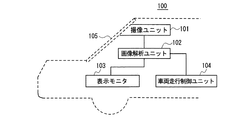•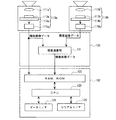•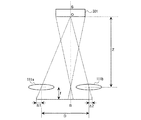•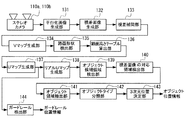•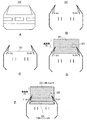•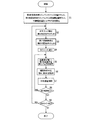•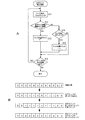•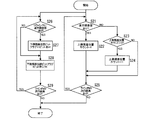•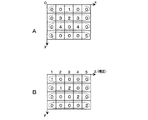•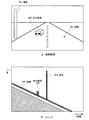•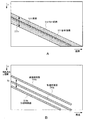•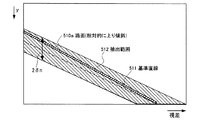•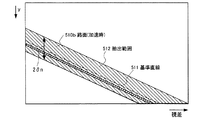•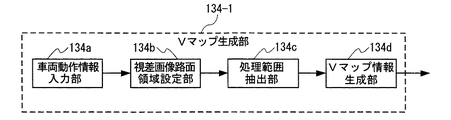•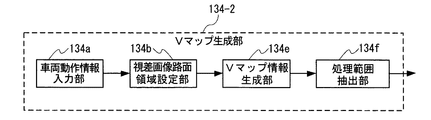•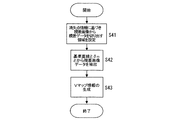•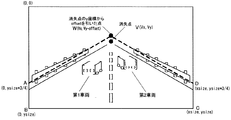•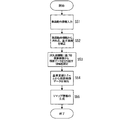•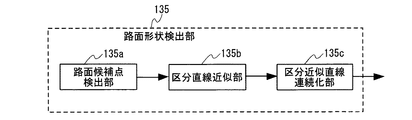•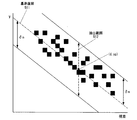•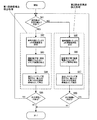••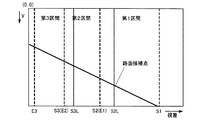•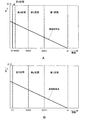•••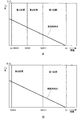•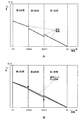•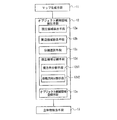••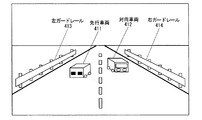•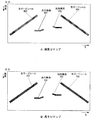•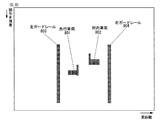•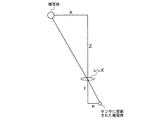•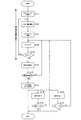•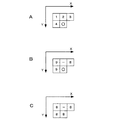•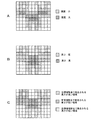•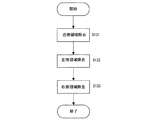•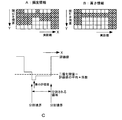•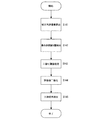•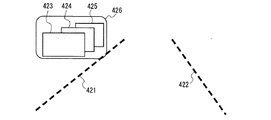•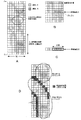•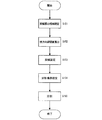•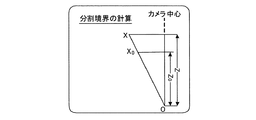•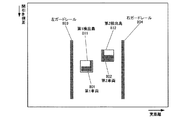•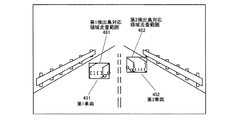•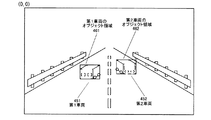•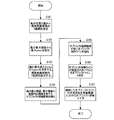•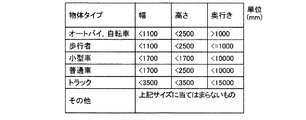•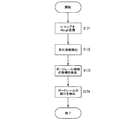•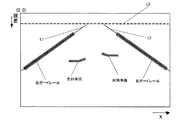•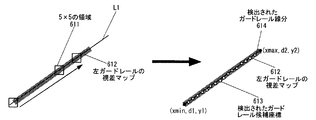•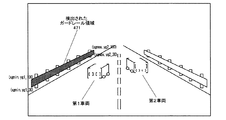•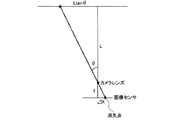•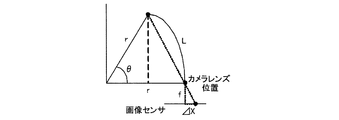•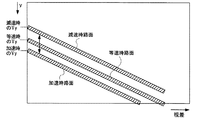•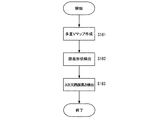•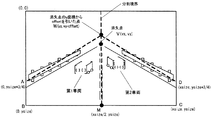•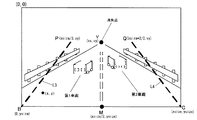•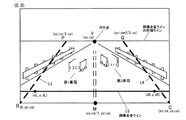•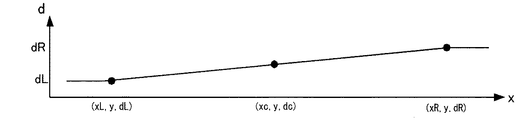•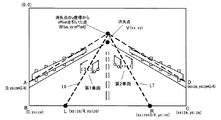••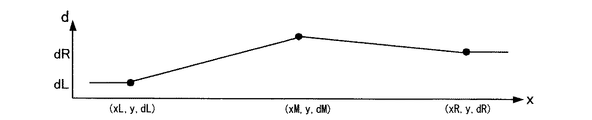•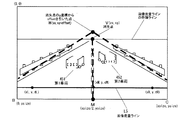## Classifications

• GPHYSICS
• G06COMPUTING; CALCULATING; COUNTING
• G06KRECOGNITION OF DATA; PRESENTATION OF DATA; RECORD CARRIERS; HANDLING RECORD CARRIERS
• G06K9/00Methods or arrangements for reading or recognising printed or written characters or for recognising patterns, e.g. fingerprints
• G06K9/00624Recognising scenes, i.e. recognition of a whole field of perception; recognising scene-specific objects
• G06K9/00791Recognising scenes perceived from the perspective of a land vehicle, e.g. recognising lanes, obstacles or traffic signs on road scenes
• GPHYSICS
• G06COMPUTING; CALCULATING; COUNTING
• G06KRECOGNITION OF DATA; PRESENTATION OF DATA; RECORD CARRIERS; HANDLING RECORD CARRIERS
• G06K9/00Methods or arrangements for reading or recognising printed or written characters or for recognising patterns, e.g. fingerprints
• G06K9/36Image preprocessing, i.e. processing the image information without deciding about the identity of the image
• G06K9/46Extraction of features or characteristics of the image
• GPHYSICS
• G06COMPUTING; CALCULATING; COUNTING
• G06TIMAGE DATA PROCESSING OR GENERATION, IN GENERAL
• G06T11/002D [Two Dimensional] image generation
• G06T11/20Drawing from basic elements, e.g. lines or circles
• G06T11/206Drawing of charts or graphs
• GPHYSICS
• G06COMPUTING; CALCULATING; COUNTING
• G06TIMAGE DATA PROCESSING OR GENERATION, IN GENERAL
• G06T7/00Image analysis
• G06T7/20Analysis of motion
• GPHYSICS
• G06COMPUTING; CALCULATING; COUNTING
• G06TIMAGE DATA PROCESSING OR GENERATION, IN GENERAL
• G06T7/00Image analysis
• G06T7/70Determining position or orientation of objects or cameras
• HELECTRICITY
• H04ELECTRIC COMMUNICATION TECHNIQUE
• H04NPICTORIAL COMMUNICATION, e.g. TELEVISION
• H04N13/00Stereoscopic video systems; Multi-view video systems; Details thereof
• H04N13/20Image signal generators
• H04N13/204Image signal generators using stereoscopic image cameras
• GPHYSICS
• G06COMPUTING; CALCULATING; COUNTING
• G06KRECOGNITION OF DATA; PRESENTATION OF DATA; RECORD CARRIERS; HANDLING RECORD CARRIERS
• G06K9/00Methods or arrangements for reading or recognising printed or written characters or for recognising patterns, e.g. fingerprints
• G06K9/36Image preprocessing, i.e. processing the image information without deciding about the identity of the image
• G06K9/46Extraction of features or characteristics of the image
• G06K2009/4666Extraction of features or characteristics of the image regional/local feature not essentially salient, e.g. local binary pattern
• GPHYSICS
• G06COMPUTING; CALCULATING; COUNTING
• G06TIMAGE DATA PROCESSING OR GENERATION, IN GENERAL
• G06T2207/00Indexing scheme for image analysis or image enhancement
• G06T2207/10Image acquisition modality
• G06T2207/10004Still image; Photographic image
• G06T2207/10012Stereo images
• GPHYSICS
• G06COMPUTING; CALCULATING; COUNTING
• G06TIMAGE DATA PROCESSING OR GENERATION, IN GENERAL
• G06T2207/00Indexing scheme for image analysis or image enhancement
• G06T2207/10Image acquisition modality
• G06T2207/10016Video; Image sequence
• G06T2207/10021Stereoscopic video; Stereoscopic image sequence
• GPHYSICS
• G06COMPUTING; CALCULATING; COUNTING
• G06TIMAGE DATA PROCESSING OR GENERATION, IN GENERAL
• G06T2207/00Indexing scheme for image analysis or image enhancement
• G06T2207/30Subject of image; Context of image processing
• G06T2207/30248Vehicle exterior or interior
• G06T2207/30252Vehicle exterior; Vicinity of vehicle
• GPHYSICS
• G06COMPUTING; CALCULATING; COUNTING
• G06TIMAGE DATA PROCESSING OR GENERATION, IN GENERAL
• G06T2207/00Indexing scheme for image analysis or image enhancement
• G06T2207/30Subject of image; Context of image processing
• G06T2207/30248Vehicle exterior or interior
• G06T2207/30252Vehicle exterior; Vicinity of vehicle
• G06T2207/30261Obstacle
• HELECTRICITY
• H04ELECTRIC COMMUNICATION TECHNIQUE
• H04NPICTORIAL COMMUNICATION, e.g. TELEVISION
• H04N13/00Stereoscopic video systems; Multi-view video systems; Details thereof
• H04N13/20Image signal generators
• H04N13/204Image signal generators using stereoscopic image cameras
• H04N13/239Image signal generators using stereoscopic image cameras using two 2D image sensors having a relative position equal to or related to the interocular distance
• HELECTRICITY
• H04ELECTRIC COMMUNICATION TECHNIQUE
• H04NPICTORIAL COMMUNICATION, e.g. TELEVISION
• H04N13/00Stereoscopic video systems; Multi-view video systems; Details thereof
• H04N2013/0074Stereoscopic image analysis
• H04N2013/0081Depth or disparity estimation from stereoscopic image signals

## Description

The present invention relates to a three-dimensional object detection apparatus, a three-dimensional object detection method, and a three-dimensional object detection method for detecting a three-dimensional object existing in front of a moving object based on a plurality of captured images BACKGROUND OF THE INVENTION 1. Field of the Invention The present invention relates to an object detection program and a mobile device control system for controlling a device mounted on a mobile using a detection result.

In terms of vehicle safety, in the past, developments have been made on the body structure of a car and the like from the viewpoint of how to protect the pedestrian when it collides with a pedestrian or a car and how to protect the occupants. However, in recent years, with the development of information processing technology and image processing technology, technology for detecting people and vehicles at high speed has been developed. By applying these technologies, vehicles are also put on the market to automatically brake before a collision to prevent a collision. To apply braking automatically, it is necessary to accurately measure the distance to people and other vehicles. For that purpose, millimeter-wave radar, ranging with laser radar, ranging with stereo camera, etc. are put to practical use .

In order to accurately detect the three-dimensional position or size of an object such as a person or another vehicle with a stereo camera, it is necessary to detect parallax in the horizontal direction, and block matching is a method used to detect parallax. The scheme is known.

However, although the block matching method can detect with high accuracy the parallax of a part near a vertical edge or a texture, the parallax can not be detected at an edge close to a horizontal, or even if it can be detected, the accuracy is not high. .

For this reason, it has been difficult to recognize a three-dimensional object, particularly a car traveling ahead, as a single object. The reason is that a car traveling forward is a box-like object, and it can be regarded as a set of vertical lines at the left and right ends and horizontal lines connecting them, so in the block matching method, only the vertical lines at both ends are detected It is often impossible to recognize an object as two parallel objects.

As an invention corresponding to this problem, there is a forward vehicle detection device and method described in Patent Document 1. In this forward vehicle detection device and method, in order to detect a leading vehicle in the own lane and to obtain a distance, the parallax image after removing an object at the same height as the road surface has the same parallax value on the left and right When there is a pixel, the parallax value is substituted into the pixel between the left and right pixels to create an interpolated parallax image, thereby recognizing one object.

However, in the forward vehicle detection apparatus and method described in Patent Document 1, the parallax is interpolated between the car and another car running parallel to the car, a nearby sign, and another three-dimensional object, and the object is accurately detected. Problem that can not be recognized.

Further, it is known that there is a variance in the parallax value, and the proportion of the variance to the detected parallax value increases as the distance to the subject increases as the baseline length of the stereo camera decreases. For this reason, when a plurality of vehicles are traveling in tandem in front of the adjacent lane and a part of the distant vehicles is visible, the parallax values of the vehicles in front and the vehicles in back are connected, and one isolated area It may be detected as

In this case, although detection as an object is possible, it affects the calculation of the distance to the object based on the average value of the parallax values of the isolated region, and the detected distance may be larger than the distance to the object in front. If the distance to the object is not accurate, functions such as automatic emergency braking may not work properly.

As described above, the conventional forward vehicle detection apparatus and method have a problem that a plurality of objects are detected as one isolated area, and correct object detection can not be performed.

The present invention has been made to solve such a problem, and an object thereof is to obtain an image of the front of the moving direction of the moving body by a plurality of imaging means mounted on the moving body such as a vehicle. When detecting a three-dimensional position or size of a solid object existing in front of the moving direction based on a plurality of captured images and a parallax image generated from the captured images, connection of the plurality of solid objects is It is to prevent so that the exact position and size of a solid thing can be detected.

The three-dimensional object detection device according to the present invention is based on a plurality of captured images obtained by capturing an image of the front of the moving direction of the moving object by a plurality of imaging units mounted on the moving object and parallax images generated from the captured images. A three-dimensional object detection device for detecting a three-dimensional object existing in front of the moving direction,
Map generation means for generating a map representing a frequency distribution of parallax values in which the distance in the lateral direction with respect to the movement direction is associated with the distance in the movement direction based on the parallax image; Isolated area detecting means for detecting, isolated area dividing means for dividing the isolated area based on the frequency distribution in the isolated area, and solid object detecting means for detecting a three-dimensional object based on the divided isolated area And a three-dimensional object detection device.

According to the present invention, the movement is performed based on a plurality of captured images obtained by capturing an image of the front of the moving direction of the moving body by a plurality of imaging units mounted on the moving body and a parallax image generated from the captured image. When detecting the three-dimensional position and size of a three-dimensional object present in front of the direction, it is possible to prevent the connection of a plurality of three-dimensional objects and to detect the accurate position and size of the three-dimensional object.

Hereinafter, an embodiment of a mobile device control system having a three-dimensional object detection device according to the present invention will be described.

<Schematic Configuration of In-vehicle Device Control System>
FIG. 1 is a schematic view showing a schematic configuration of an in-vehicle device control system as a mobile device control system according to an embodiment of the present invention.
The on-vehicle device control system is mounted on the vehicle 100 such as a car which is a moving body, and includes an imaging unit 101, an image analysis unit 102, a display monitor 103, and a vehicle travel control unit 104. The relative height information (relatively) of the road surface (moving surface) ahead of the vehicle is obtained from the captured image data of the forward region (imaging region) of the traveling direction (moving direction) of the vehicle taken by the imaging unit 101 Information indicating the inclination state is detected, and from the detection result, the three-dimensional shape of the traveling road surface ahead of the host vehicle is detected, and control of various in-vehicle devices is performed using the detection result.

The imaging unit 101 is installed, for example, in the vicinity of a rearview mirror (not shown) of the windshield 105 of the vehicle 100. Various data such as captured image data obtained by imaging of the imaging unit 101 is input to an image analysis unit 102 as an image processing unit. The image analysis unit 102 analyzes the data transmitted from the imaging unit 101, and on the traveling road surface ahead of the vehicle with respect to the road surface portion on which the vehicle 100 is traveling (a road surface portion located directly below the vehicle). The relative height (position information) at each point is detected, and the three-dimensional shape of the traveling road surface ahead of the host vehicle is grasped.

The analysis result of the image analysis unit 102 is sent to the display monitor 103 and the vehicle travel control unit 104. The display monitor 103 displays the captured image data and the analysis result obtained by the imaging unit 101. The vehicle travel control unit 104 recognizes recognition objects such as other vehicles, pedestrians and various obstacles ahead of the host vehicle based on the recognition result of the relative inclination state of the traveling road surface by the image analysis unit 102, and Based on the warning, the driver of the vehicle 100 is notified of a warning, and driving support control such as control of the steering wheel and the brake of the vehicle is performed.

<Schematic Configuration of Imaging Unit 101 and Image Analysis Unit 102>
FIG. 2 is a schematic view showing a schematic configuration of the imaging unit 101 and the image analysis unit 102 in FIG.

The imaging unit 101 is configured of a stereo camera including two imaging units 110a and 110b as imaging means, and the two imaging units 110a and 110b are the same. The respective imaging units 110a and 110b respectively include imaging lenses 111a and 111b, sensor substrates 114a and 114b including image sensors 113a and 113b on which light receiving elements are two-dimensionally arranged, and analog signals output from the sensor substrates 114a and 114b. Electric signal (electrical signal corresponding to the amount of light received by each light receiving element on the image sensors 113a, 113b) is converted into digital electric signal, and it is composed of signal processing units 115a, 115b for generating and outputting captured image data ing. Luminance image data and parallax image data are output from the imaging unit 101.

The imaging unit 101 also includes a processing hardware unit 120 configured of an FPGA (Field-Programmable Gate Array) or the like. In order to obtain parallax images from the luminance image data output from the imaging units 110a and 110b, the processing hardware unit 120 calculates the parallax values of corresponding image portions between the imaged images captured by the imaging units 110a and 110b. A disparity calculating unit 121 is provided as a disparity image information generating unit to calculate.

The parallax value referred to here is a comparison image with respect to an image portion on a reference image corresponding to the same point in the imaging region, with one of the images taken by each of the imaging units 110a and 110b as a reference image and the other as a comparison image. The positional displacement amount of the upper image portion is calculated as the parallax value of the image portion. By using the principle of triangulation, it is possible to calculate the distance from the parallax value to the same point in the imaging region corresponding to the image portion.

FIG. 3 is a diagram for explaining the principle of calculating the distance from the parallax value by using the principle of triangulation. In the figure, f is the focal length of each of the imaging lenses 111a and 111b, and D is the distance between the optical axes. Further, Z is the distance from the imaging lenses 111a and 111b to the subject 301 (the distance in the direction parallel to the optical axis). In this figure, the imaging positions in the left and right images with respect to the point O on the subject 301 are respectively Δ1 and Δ2 from the imaging center. The parallax value d at this time can be defined as d = Δ1 + Δ2.

It returns to the explanation of FIG. The image analysis unit 102 is formed of an image processing board or the like, and has storage means 122 such as a RAM or ROM for storing luminance image data and parallax image data output from the imaging unit 101, recognition processing of an identification target, A CPU (Central Processing Unit) 123 that executes a computer program for performing parallax calculation control and the like, a data I / F (interface) 124, and a serial I / F 125 are provided.

The FPGA that configures the processing hardware unit 120 performs processing that requires real-time processing on image data, such as gamma correction, distortion correction (parallelization of right and left captured images), and parallax calculation by block matching to generate a parallax image. And the like, and performs processing such as writing out in the RAM of the image analysis unit 102. The CPU of the image analysis unit 102 is responsible for control of the image sensor controller of each of the imaging units 110a and 110b and overall control of the image processing substrate. Also, a three-dimensional object detection program for executing three-dimensional shape detection processing of a road surface, detection processing of guardrails and other various objects (identification target objects) is loaded from ROM, and luminance image data and parallax images stored in RAM are stored. Data is input to execute various processing, and the processing result is output from the data I / F 124 and the serial I / F 125 to the outside. At the time of execution of such processing, using the data I / F 124, vehicle operation information such as the vehicle speed, acceleration (acceleration mainly generated in the front and rear direction of the vehicle) of the vehicle 100, steering angle, yaw rate etc. It can also be used as a processing parameter. The data output to the outside is used as input data for performing control (brake control, vehicle speed control, warning control, etc.) of various devices of the host vehicle 100.

<Overview of Object Detection Processing>
FIG. 4 is a functional block diagram for explaining object detection processing implemented by the processing hardware unit 120 and the image analysis unit 102 in FIG. Hereinafter, an object detection process in the present embodiment will be described.

Luminance image data is output from the two imaging units 110a and 110b constituting the stereo camera. At this time, when the imaging units 110a and 110b are in color, color luminance conversion to obtain a luminance signal (Y) from the RGB signals is performed using, for example, the following formula .
Y = 0.3R + 0.59G + 0.11B ... Formula 

<< Parallelized image generation processing >>
First, parallelized image generation processing is performed on the luminance image data by the parallelized image generation unit 131. In this parallelized image generation process, luminance image data (reference image) output from each of the imaging units 110a and 110b is obtained based on the distortion of the optical system in the imaging units 110a and 110b and the relative positional relationship between the left and right imaging units 110a and 110b. And the comparison image) are transformed to be an ideal collimated stereo image obtained when two pinhole cameras are mounted in parallel. In this calculation, the distortion amount at each pixel is calculated using polynomials Δx = f (x, y) and Δy = g (x, y), and the calculation results are used to calculate the distortion amount from each imaging unit 110a, 110b. Each pixel of the luminance image data (reference image and comparison image) to be output is converted. The polynomial is based, for example, on a fifth order polynomial with respect to x (horizontal position of the image) and y (longitudinal position of the image).

Parallax image generation processing
After the parallelization image processing is performed as described above, next, parallax image generation processing for generating parallax image data (parallax image information) in the parallax image generation unit 132 configured by the parallax calculation unit 121 (FIG. 2) I do. In the parallax image generation process, first, luminance image data of one of the two imaging units 110a and 110b is used as reference image data, and luminance image data of the other imaging unit 110b is used as comparison image data. The disparity between the two is calculated using this to generate and output disparity image data. The parallax image data represents a parallax image in which pixel values corresponding to the parallax value d calculated for each image portion on the reference image data are represented as pixel values of the respective image portions.

Specifically, the parallax image generation unit 132 defines a block including a plurality of pixels (for example, 16 pixels × 1 pixel) centered on one target pixel for a row of reference image data. On the other hand, in the same row in the comparison image data, a block of the same size as the block of the defined reference image data is shifted by one pixel in the horizontal line direction (X direction) to indicate the feature of the pixel value of the block defined in the reference image data. A correlation value indicating a correlation between the feature amount and the feature amount indicating the feature of the pixel value of each block in the comparison image data is calculated. Then, based on the calculated correlation value, a matching process is performed to select a block of comparison image data that is most correlated with the block of reference image data among the blocks of comparison image data. Thereafter, the amount of positional deviation between the target pixel of the block of the reference image data and the corresponding pixel of the block of the comparison image data selected in the matching process is calculated as the parallax value d. It is possible to obtain parallax image data by performing such a process of calculating the parallax value d for the entire area of the reference image data or a specific area.

For example, the value (brightness value) of each pixel in the block can be used as the feature amount of the block used for the matching process, and the correlation value can be, for example, the value of each pixel in the block of the reference image data (brightness A sum of absolute values of differences between the values) and the values (brightness values) of the respective pixels in the block of the comparison image data respectively corresponding to these pixels can be used. In this case, it can be said that the block with the smallest total sum is most correlated.

When the matching processing in the parallax image generation unit 132 is realized by hardware processing, for example, SSD (Sum of Squared Difference), ZSSD (Zero-mean Sum of Squared Difference), SAD (Sum of Absolute Difference), ZSAD (Sum of Absolute Difference). A method such as Zero-mean Sum of Absolute Difference can be used. In addition, since only the parallax value in a pixel unit can be calculated in the matching process, it is necessary to use an estimated value when a parallax value of a sub-pixel level less than one pixel is required. As the estimation method, for example, an equiangular linear method, a quadratic curve method or the like can be used. However, since an error occurs in the estimated disparity value at the sub-pixel level, it is possible to use EEC (estimated error correction) or the like for reducing the estimated error.

<< Overview of parallax image interpolation processing >>
After performing parallax image generation processing, next, in the parallax interpolation unit 133 configured by the image analysis unit 102, parallax image interpolation processing is performed to generate an interpolated parallax image. FIG. 5 is a diagram for describing an outline of parallax image interpolation processing. Here, FIG. 5A is a captured image, and FIG. 5B is a parallax image. 5C to 5E are diagrams for explaining conditions for executing parallax image interpolation processing.

The parallax image 320 shown in FIG. 5B is generated by the parallax image generator 132 from the captured image (luminance image) 310 of the vehicle shown in FIG. 5A. Since the parallax value d is the degree of positional displacement in the horizontal direction, the parallax can not be calculated at the horizontal edge portion of the captured image 310 or the portion with a small change in luminance, so the vehicle can not be recognized as one object.

Therefore, in the parallax interpolation unit 133, two pixels on the same line of the parallax image, that is, two pixels of the same Y coordinate (vertical direction of the image) shown in FIG. 5B, based on the following five judgment conditions a to e. P1 (disparity value D1) and P2 (disparity value D2) are interpolated.

a: The actual distance between two points is shorter than a predetermined length (first determination condition).
Assuming that the distance corresponding to the parallax value D1 is Z1 and the distance on the image between the pixels P1 and P2 is PX, an approximate actual distance RZ between two points is f, the focal distance of the stereo camera is “RZ = It can be calculated by “Z1 / f * PX”. If RZ is within a predetermined value (width corresponding to one passenger car: 1900 mm), this condition is satisfied.

b: There is no other disparity value between the two points (second determination condition).
That is, no parallax value exists in the pixel on the line 321 connecting the pixel P1 and the image P2 in FIG. 5C.

c: The difference in depth between two points (difference in distance in the forward direction of the vehicle 100) is smaller than the threshold set according to one of the distances, or the difference in depth between two points is measured at one of the distances It is smaller than the threshold set according to the distance accuracy (third determination condition).

Here, the distance Z1 is calculated from the parallax value D1 of the pixel P1 on the left side. The distance measurement accuracy of the stereo method, in particular, the distance measurement accuracy by block matching depends on the distance. That is, the accuracy is, for example, the distance ± 10%. Therefore, if the distance measurement accuracy is 10%, the threshold value of the depth difference is set to 20% of Z1 (= Z1 * 0.2).

d: A horizontal edge is present at a position higher than two points and not more than a predetermined height (for example, 1.5 m: corresponding to the vehicle height) (fourth determination condition).
That is, as shown in FIG. 5D, it is determined, for example, whether or not a predetermined number or more of horizontal edges exist in an area 322 having a height within 2 to 1.5 m. The number of pixels PZ corresponding to a height of 1.5 m is calculated from the distance Z1 calculated from the parallax value D1 of the pixel P1 and the focal distance f of the stereo camera according to the formula “PZ = 1.5 m * f / Z1”. be able to.

Here, "when there is a horizontal edge" means that there is a horizontal edge in a region 322 above the pixel (target pixel) between the pixels P1 and P2. That is, the value at the pixel position of the line buffer of the edge position count described later is 1 or more and PZ or less.

Then, after performing one line of horizontal edge detection processing (step S2 in FIG. 6, FIG. 7A) to be described later, when trying to interpolate between pixels P1 and P2 in parallax interpolation of the next line The fourth determination condition is true when the presence of an edge is larger than 1/2 of the number of pixels between the pixels P1 and P2.
The assumed scene is the roof 323 of the vehicle. If the horizontal edge is continuous, interpolation is performed if the difference between the parallax values D1 and D2 of the pixels P1 and P2 is equal to or less than a predetermined value.

e: There is no disparity information (far disparity value) farther from the two points in the upper and lower vicinity between the two points (fifth determination condition).
The distant parallax information is a parallax value corresponding to a distant distance of 20% or more of the larger value of the distances Z1 and Z2 obtained from the parallax values D1 and D2.

That is, for example, as shown in FIG. 5E, in the region 322 at a position higher than the pixels P1 and P2 (within 1.5 m on the upper side; within PZ in terms of the number of pixels), or in the region 324 within 10 lines below the region 322, The case where far parallax is present in the vertical direction of each pixel between the pixels P1 and P2 is counted for all pixels between the pixels P1 and P2. The fifth determination condition is true when the sum is equal to or greater than a predetermined value (for example, 2).

Here, “when a pixel between P1 and P2 has a far parallax” means that the upper parallax position count described later has a value of 1 or more and PZ or less, or each bit of the lower parallax position bit flag described later If one of them is standing.
The case where the fifth determination condition is false is a case where a distant parallax is present in the vicinity of the line to be interpolated, that is, a case where a distant object is viewed. In that case, parallax interpolation is not performed.

<< Flow of parallax image interpolation processing >>
Next, disparity interpolation processing will be described in detail.
FIG. 6 is a flowchart showing the entire flow of parallax image interpolation processing.
First, the fourth determination condition line buffer (edge position count) and the fifth determination condition line buffer (upper disparity position count, lower disparity position bit flag) are initialized (step S1).

Here, the edge position count is a counter set in the line buffer in order to hold, in pixel units, information representing how many horizontal edges above the line to be subjected to disparity interpolation. Further, the upper parallax position count is a counter set in the line buffer to hold information indicating how many distant parallax values exist in the area 322 above the line subjected to parallax interpolation. The lower parallax position bit flag is a counter set in the line buffer to hold information indicating that there is a far parallax value within the lower 10 lines (in the area 324) of the line subjected to parallax interpolation. The lower parallax position bit flag is prepared by the number of pixels of one line of 11-bit flags.

Next, a horizontal edge of a certain line is detected for the fourth determination condition (step S2). FIG. 7 is a diagram for describing the details of step S2 (horizontal edge detection processing). Here, FIG. 7A is a flowchart showing an algorithm of horizontal edge detection processing, and FIG. 7B is a view showing an example of edge position count and change of the value thereof.

First, the intensity image is processed with a Sobel filter to obtain the strengths of vertical edges and horizontal edges (step S11), and it is determined whether the horizontal edge strength exceeds twice the vertical edge strength (step S11). S12).

When the horizontal edge strength exceeds twice the vertical edge strength (step S12: YES), it is determined that there is a horizontal edge, and the edge position count is set to 1 (step S13). On the other hand, if the horizontal edge strength is equal to or less than twice the vertical edge strength (step S12: NO), it is judged that there is no horizontal edge, and it is judged whether the edge position count is larger than 0 (step S14). When it is larger than 0 (step S14: YES), the edge position count is incremented by one (step S15). If it is determined in step S14 that the edge position count is 0 (step S14: NO), the edge position count is not updated.

After updating the edge position count value in steps S13 and S15 based on the presence / absence of the horizontal edge and the edge position count value, or after determining that the edge position count is 0 in step S14 (step S14: NO), step S16 To determine the presence or absence of the next pixel in the line.

When there is a next pixel (step S16: YES), the process proceeds to step S11, and steps S11 to S15 are repeated. When there is no next pixel (step S16: NO), the horizontal edge detection process for one line shown in this figure ends.

As shown in FIG. 7A, the initial value of the edge position count corresponding to each pixel is 0 initialized in step S1. When a horizontal edge starts to be found in a certain line, the edge position count is set to 1 in step S13. FIG. 7B shows that horizontal edges have been detected for six pixels excluding the central two pixels and the four pixels at both ends out of a total of 12 pixels. The next line indicates that horizontal edges have been detected for eight pixels excluding the four pixels at both ends. In the next line, since the horizontal edge is not detected, the edge position count is incremented to 2 in step S15.

When a horizontal edge is detected in each subsequent line, the value of the edge position count becomes 1, and the edge position count is incremented by 1 unless a horizontal edge is detected. Therefore, the value of the edge position count corresponding to each pixel indicates the number of lines on which horizontal interpolation is to be performed on the parallax interpolation.

When the horizontal edge detection process for one line is completed, the far parallax value is detected for the fifth determination condition (step S3). FIG. 8 is a flowchart showing an algorithm of step S3 (far disparity value detection processing).

The processing for the upper area 322 in FIG. 5E (hereinafter, upper far parallax value detection processing) and the processing for the lower area 324 (hereinafter, lower lower parallax value detection processing) are performed in parallel. The disparity value detection process and the lower far disparity value detection process will be individually described in this order.

In the upper side far parallax value detection process, first, the presence or absence of the far parallax value is determined (step S21). As a result of the judgment, when there is (step S21: YES), the upper parallax position count is set to 1 (step S22), and when it is not (step S21: NO), whether the upper parallax position count is larger than 0 or not It judges (step S23). Then, when it is larger than 0 (step S23: YES), the upper parallax position count is incremented by one (step S24).

After updating the value of the upper parallax position count in step S22 or S24 based on the presence of the far parallax value and the value of the upper parallax position count at that time, or after determining that the upper parallax position count is 0 in step S23 (step S23: NO) Go to step S25, and determine the presence or absence of the next pixel in the line.

When there is a next pixel (step S25: YES), the process proceeds to step S21, and steps S21 to S24 are repeated. If there is no next pixel (step S25: NO), the upper distant disparity value detection process for one line shown in this figure ends.

That is, in the upper side far disparity value detection process, the horizontal edge in the process shown in FIG. 7A is changed to the far disparity value.

In the lower distant parallax value detection process, first, it is determined whether there is a distant parallax value in the lower 11th line (step S26). As a result of determination, if there is (step S26: YES), the 11th bit of the lower parallax position bit flag is set to 1 (step S27), and then the lower parallax position bit flag is shifted 1 bit to the right (step S27) S28). As a result of the determination, if there is not (step S26: NO), the lower disparity position bit flag is shifted to the right by one bit as it is. Thereby, the position of the lower far parallax value in the closest line in the lower 10 lines of the pixels between the two pixels (P1, P2) can be determined.

While there is the next pixel in the line (step S29: YES), steps S26 to S28 are repeated, and if there is no more (step S29: NO), the processing for detecting the lower distant parallax value for one line shown in this figure ends. Do.

When the far parallax value detection process for one line is completed, the process proceeds to the next line (step S4), and two points satisfying the first to third determination conditions are set (step S5). Next, it is checked whether or not the fourth and fifth determination conditions are satisfied at the two points set in step S5 (step S6), and if it is satisfied, the parallax value is interpolated (step S7). At this time, an average value of disparity values at two points is set as a disparity value between two points.

While there is a pixel for which the parallax value is to be interpolated (step S8: YES), steps S5 to S7 are repeated, and if there is no more (step S8: NO), the process proceeds to step S9 to determine the presence or absence of the next line. Then, while there is a next line (step S9: YES), steps S2 to S8 are repeated, and when there is no more (step S9: NO), the parallax interpolation process shown in this figure ends.

Here, the horizontal edge detection processing (step S2 in FIG. 6, steps S11 to S16 in FIG. 7) and the lower far parallax value detection processing (steps S26 to S29 in FIG. 8) will be supplemented.

In the horizontal edge detection processing, for example, horizontal edge detection processing is performed from the line at the upper end of the luminance image shown in FIG. 5A, and it is assumed that the horizontal edge is detected for the first time in the line corresponding to the roof 323 shown in FIG.

In this case, the value of the edge position count remains at the initial value 0 during the period from the line at the upper end of the luminance image to the line immediately above the line corresponding to the roof 323 for the horizontal edge detection process (step S12) : NO → S14: NO). Therefore, after the horizontal edge detection process, the far parallax value detection process (step S3) is performed, the process proceeds to the next line in step S4, and two points can be set in step S5. The fourth determination condition is not satisfied because it does not correspond to “when there is a horizontal edge” when determining whether or not there is a horizontal edge.

When a horizontal edge is detected in the line corresponding to the roof 323 (step S12: YES), the value of the edge position count corresponding to the pixel where the horizontal edge is detected becomes 1 (step S13). At this time, the determination processing (step S6) of the fourth determination condition for the line next to the line corresponding to the roof 323 corresponds to "when there is a horizontal edge". Therefore, the fourth determination condition is satisfied if “the case where a horizontal edge exists between the pixels P1 and P2 is larger than 1⁄2 of the number of pixels between the pixels P1 and P2” in the fourth determination condition is satisfied. Become. The value 1 of the edge position count at this time indicates that there is a horizontal edge on one line of the two points (pixels P1 and P2), that is, the line corresponding to the roof 323.

Furthermore, when the horizontal edge is not detected in each line below the line following the line corresponding to the roof 323 (step S12: NO), the edge corresponding to the pixel in which the horizontal edge is detected in the line corresponding to the roof 323 The position count value is incremented by one each time the horizontal edge detection process is performed (step S14: YES → S15). During this time, it corresponds to “when there is a horizontal edge” in the fourth determination condition. Therefore, the fourth determination condition is satisfied if “the case where a horizontal edge exists between the pixels P1 and P2 is larger than 1⁄2 of the number of pixels between the pixels P1 and P2” in the fourth determination condition is satisfied. Become. The edge position count value, which is incremented by one each time horizontal edge detection processing is performed, is set to two points (pixel P1, P2 and P4) set in step S5 on the line next to the line on which the horizontal edge detection processing is performed. The number of lines from P2) to the line corresponding to the roof 323 is shown.

In the lower far parallax value detection process, for example, the lower far parallax value is detected for the first time at a line 325 shown in FIG. 5E as a result of the far parallax value detection process being performed from the line at the upper end of the luminance image shown in FIG. Do.

In this case, all 11 bits of the lower disparity position bit flag remain 0 while the target of the lower far disparity value detection processing is from the line at the upper end of the luminance image to the line immediately above the line 325 (0 Step S26: NO → S28). For this reason, after the far parallax value detection processing, the process proceeds to the next line in step S4, and two points can be set in step S5. When it is determined whether the fifth determination condition is satisfied in step S6, the fifth determination condition In the case where "a certain pixel between P1 and P2 has a distant parallax" in the above, it does not correspond to "one of each bit of the lower parallax position bit flag is set".

When the lower far parallax value is detected on the line 325, the 11th bit of the lower parallax position bit flag becomes 1 (step S26: YES → S27), and the 10th bit becomes 1 (step S28). ). At this time, in the determination processing of the fifth determination condition for the line following the line 325 (step S6), it corresponds to "one of each bit of the lower parallax position bit flag is set". The fact that the 10th bit of the lower disparity position bit flag at this time is 1 indicates that there is a far disparity value below 10 lines of 2 points (pixels P1 and P2).

Furthermore, when the lower distant parallax value is not detected in each line below the line following the line 325 (step S26: NO), the lower parallax position is detected each time the target line of the lower distant parallax value detection process goes down. Bit flag 1 shifts to the right. Therefore, for example, when the eighth bit of the lower parallax position bit flag is 1, it indicates that there is a lower far parallax value below 8 lines of 2 points (pixels P1 and P2).

Here, an advantage of the parallax image interpolation process according to the present embodiment will be described.
If it is going to interpolate the parallax value of two points (pixel P1, P2) in FIG. 5B, following four judgment steps of (i)-(vi) will be needed.
(A) It is determined whether or not the parallax values of the two points are close.
(B) It is determined whether or not there is a horizontal edge within 1.5 m above the two points.
(C) It is determined whether there is a far disparity value below the horizontal edge.
(D) It is determined whether there is a far disparity value below the two points.

Then, when it is determined that the parallax is close, the execution time can not be read due to the content shown in the image when the process of detecting whether there is a horizontal edge and the far parallax value is executed every time. It may also take very long processing time.

On the other hand, in the present embodiment, each line is scanned in synchronization with determining whether the parallax value is close to detect the presence or absence of the horizontal edge and the presence or absence of the distant parallax. The processing time is constant with almost no change even if an image of various contents is input. Therefore, estimation of the execution time becomes easy, which is effective when constructing an apparatus or system that performs real-time processing. Further, even when high speed is required, the processing time can be significantly reduced by thinning out the pixels to be processed.

<< V map generation process >>
After the parallax image interpolation process is performed as described above, next, the V map generation unit 134 performs the V map generation process of generating the V map. Each piece of parallax pixel data included in parallax image data is indicated by a set (x, y, d) of an x-direction position, a y-direction position, and a parallax value d. This is converted into three-dimensional coordinate information (d, y, f) with d set on the X axis, y on the Y axis, and frequency f on the Z axis, or this three-dimensional coordinate information (d, y, f) , And three-dimensional coordinate information (d, y, f) limited to information exceeding a predetermined frequency threshold is generated as disparity histogram information. The disparity histogram information of the present embodiment is composed of three-dimensional coordinate information (d, y, f), and a distribution of this three-dimensional histogram information in an X-Y two-dimensional coordinate system is a V map (disparity histogram map Call it).

Specifically, the V map generation unit 134 calculates a disparity value frequency distribution for each row region of disparity image data obtained by dividing the image into a plurality of pieces in the vertical direction. Information indicating this disparity value frequency distribution is disparity histogram information.

FIG. 9 is a diagram for describing parallax image data and a V map generated from the parallax image data. Here, FIG. 9A is a diagram showing an example of the parallax value distribution of the parallax image, and FIG. 9B is a diagram showing a V map showing the parallax value frequency distribution for each row of the parallax image of FIG. 9A.

When parallax image data having a parallax value distribution as shown in FIG. 9A is input, the V map generation unit 134 calculates a parallax value frequency distribution which is a distribution of the number of data of each parallax value for each row, This is output as disparity histogram information. On the two-dimensional orthogonal coordinate system, taking the information of the disparity value frequency distribution of each row obtained in this way on the Y axis as the y-direction position on the parallax image (the position in the vertical direction of the captured image) V can be obtained as shown in FIG. 9B. This V map can also be expressed as an image in which pixels having pixel values according to the frequency f are distributed on the two-dimensional orthogonal coordinate system.

FIG. 10 is a view showing an image example of a captured image as a reference image captured by one imaging unit and a V map corresponding to the captured image. Here, FIG. 10A is a photographed image, and FIG. 10B is a V map. That is, the V map shown in FIG. 10B is generated from the photographed image as shown in FIG. 10A. In the V map, since parallax is not detected in the area below the road surface, parallax is not counted in the hatched area A.

In the example of an image shown to FIG. 10A, the road surface 401 in which the own vehicle is drive | running | working, the preceding vehicle 402 which exists in front of the own vehicle, and the telephone pole 403 which exists out of the road are shown. Further, in the V map shown in FIG. 10B, there are a road surface 501, a leading vehicle 502, and a telephone pole 503 corresponding to the image example.

This image example is a virtual reference obtained by extending the surface in front of the host vehicle relatively flat, ie, the surface in front of the host vehicle parallel to the road surface portion directly below the host vehicle to the front of the host vehicle It corresponds to the road surface (virtual reference movement surface). In this case, in the lower part of the V map corresponding to the lower part of the image, the high frequency points are distributed in a substantially straight line with an inclination such that the parallax value d becomes smaller toward the upper side of the image. Pixels showing such a distribution are present at approximately the same distance in each row on the parallax image and have the highest occupancy rate, and pixels that project an object of identification whose distance increases continuously as they move upward in the image It can be said that

Since the imaging unit 110a captures an area in front of the host vehicle, as shown in FIG. 10A, the parallax value d of the road surface decreases toward the upper side of the image as shown in FIG. 10A. Further, in the same line (horizontal line), pixels projecting the road surface have substantially the same parallax value d. Therefore, the high-frequency points distributed in a substantially straight line on the V map correspond to the features possessed by the pixels projecting the road surface (moving surface). Therefore, it is possible to estimate that the pixels of the point distributed on the approximate straight line obtained by straight line approximation of the high frequency points on the V map or in the vicinity thereof are the pixels showing the road surface with high accuracy. Further, the distance to the road surface portion shown in each pixel can be determined with high accuracy from the parallax value d of the corresponding point on the approximate straight line.

Here, when a high frequency point on the V map is subjected to linear approximation, the range to which the point included in the linear approximation processing is to be determined largely influences the accuracy of the processing result. That is, the wider the range included in the straight line approximation process, the more points that do not correspond to the road surface are included, which lowers the processing accuracy, and the narrower the range included in the straight line approximation process, the number of points corresponding to the road surface There is also a result that the processing accuracy is reduced. So, in this embodiment, the parallax histogram information part made into the object of the straight line approximation processing mentioned below is extracted as follows.

FIG. 11 is a diagram showing a V map for explaining the extraction range.
The extraction condition in the present embodiment is a parallax corresponding to a virtual reference road surface (virtual reference movement surface) obtained by extending a plane parallel to the road surface portion 510 directly below the own vehicle ahead of the own vehicle forward to the own vehicle The condition is that it falls within a predetermined extraction range 2δ n which is determined based on the relationship between the value d and the vertical position y of the image. The relationship between the parallax value d corresponding to the reference road surface and the vertical position y of the image is indicated by a straight line (hereinafter referred to as a "reference straight line") 511 on the V map, as shown in FIG. In the present embodiment, a range of ± δ in the vertical direction of the image around the reference straight line 511 is defined as the extraction range 512. The extraction range 512 is set so as to include the variation range of the actual road surface V map element (d, y, f) which changes with the situation.

Specifically, for example, when the road surface ahead of the host vehicle is relatively uphill, the road surface image portion (moving surface image area) displayed in the captured image than in the case where the road surface is relatively flat. Spreads to the top of the image. Moreover, when the road surface image portions displayed at the same image vertical direction position y are compared, the parallax value d is larger in the case of a relatively upward slope than in the case of a relatively flat surface. In this case, the V map elements (d, y, f) are positioned approximately on the upper side with respect to the reference straight line 511 on the V map and have a large inclination (absolute value), as shown in FIG. Will be shown. In the present embodiment, the V map element (d, y, f) of the road surface falls within the extraction range 512 if the relative upward slope of the road surface 510 a ahead can be assumed.

Also, for example, when the road surface ahead of the host vehicle is relatively downward inclined, the V map element (d, y, f) is located below the reference straight line on the V map and The slope (absolute value) shows a small straight line. In the present embodiment, the V map element (d, y, f) of the road surface falls within the extraction range if the relative downward slope on the road surface ahead can be assumed.

Further, for example, during acceleration in which the host vehicle 100 is accelerating, a load is applied to the rear of the host vehicle 100, and the host vehicle is oriented such that the front of the host vehicle is directed vertically upward. In this case, the road surface image portion (moving surface image area) displayed in the captured image is shifted downward as compared to the case where the speed of the vehicle 100 is constant. In this case, the V map element (d, y, f) is a straight line located approximately below the reference straight line 511 and substantially parallel to the reference straight line on the V map, as shown in FIG. Will be shown. In the present embodiment, the V map elements (d, y, f) of the road surface 510 b fall within the extraction range 512 if acceleration of the vehicle 100 can be assumed.

In addition, for example, at the time of deceleration at which the host vehicle 100 is decelerating, a load is applied to the front of the host vehicle 100, and the host vehicle has a posture in which the host vehicle front is directed downward in the vertical direction. . In this case, the road surface image portion (moving surface image area) displayed in the captured image is shifted to the upper side of the image, as compared with the case where the speed of the vehicle 100 is constant. In this case, the V map elements (d, y, f) are located approximately on the upper side with respect to the reference straight line on the V map, and indicate a straight line substantially parallel to the reference straight line. In the present embodiment, the V map element (d, y, f) of the road surface falls within the extraction range 512 if the deceleration of the host vehicle 100 can be assumed.

In the present embodiment, the extraction range 512 for detecting the road surface is the center of the V map extraction range 512 by moving the virtual road surface straight line 511 up and down according to the up and down of the road, that is, the acceleration / deceleration. It is possible to perform road surface data extraction and approximation under optimum conditions at all times. As a result, δn can be reduced, and the extraction range 512 of the V map can be narrowed, which leads to shortening of the processing time.

The degree to which the reference straight line 511 is moved up and down according to the acceleration / deceleration of the vehicle is a relationship formula that creates a correspondence table between the output of the acceleration sensor of the vehicle and the fluctuation of the reference straight line 511 at that time by experiment. Can be determined for each vehicle.

However, it is common to all models to lower the reference straight line 511 (increase the intercept) during acceleration and raise the reference straight line 511 during deceleration (common to all vehicle types), and in fact, reference how much according to the acceleration value It corresponds by changing the intercept of the straight line 511 or having a small conversion table.

The y coordinate Vy of the vanishing point fluctuates with the fluctuation of the intercept. An area at the time of creating a multiplex V map to be described later fluctuates according to the fluctuation of the vanishing point, and it becomes possible to more accurately reflect the road surface parallax data on the V map. The details of the vanishing point will be described later.

<< Internal configuration of V map generation unit >>
FIG. 14 is a processing block diagram showing an example of the internal configuration of the V map generation unit in FIG. The V map generation unit 134-1 includes a vehicle operation information input unit 134a, a parallax image road surface area setting unit 134b, a processing range extraction unit 134c, and a V map information generation unit 134d.

When receiving the parallax image data output from the parallax interpolation unit 133, the V map generation unit 134-1 first acquires vehicle operation information including acceleration information of the host vehicle 100 in the vehicle operation information input unit 134a. The vehicle operation information input to the vehicle operation information input unit 134a may be acquired from the device mounted on the host vehicle 100, or the imaging unit 101 is mounted with vehicle operation information acquisition means such as an acceleration sensor, You may acquire from a vehicle operation | movement information acquisition means.

When vehicle operation information is acquired in this manner, next, in the parallax image road surface area setting unit 134b, a predetermined road surface image candidate area (a part of a captured image) is obtained with respect to parallax image data acquired from the parallax interpolation unit 133 Set a moving surface image candidate area). In this setting, an image area excluding an area where the road surface is not projected within the range of assumed conditions is set as a road image candidate area. As a specific setting method, a predetermined fixed image area may be set as the road surface image candidate area, but in the present embodiment, based on the vanishing point information indicating the vanishing point of the road surface in the captured image. Set the road surface image candidate area.

After setting the road surface image candidate area in this manner, next, in the processing range extraction unit 134c, the extraction condition described above is selected from the parallax image data in the road surface image candidate area set by the parallax image road surface area setting unit 134b. A process of extracting the satisfied parallax pixel data (parallax image information component) is performed. That is, parallax pixel data having a parallax value d belonging to a range of ± δn in the vertical direction of the image centering on the reference straight line 511 on the V map and the vertical position y of the image are extracted. After extracting parallax pixel data that satisfies the extraction conditions in this manner, the V map information generation unit 134 d extracts parallax pixel data (x, y, d) extracted by the processing range extraction unit 134 c as V map elements (d, y). , F) to generate V map information.

In the above description, the parallax image data corresponding to the road surface image portion is distinguished from the parallax image data portion not corresponding to the road surface image portion in the processing range extraction unit 134c of the previous stage where the V map information generation unit 134d generates the V map information. Although an example of extracting a part has been described, similar extraction processing may be performed at a stage after generating V map information as follows.

FIG. 15 is a processing block diagram in the V map generation unit 134 in an example in which extraction processing is performed at a stage after generation of V map information. The V map generation unit 134-2 includes a vehicle operation information input unit 134a, a parallax image road surface area setting unit 134b, a V map information generation unit 134e, and a processing range extraction unit 134f.

After the V map generation unit 134-2 sets the road surface image candidate area in the parallax image road surface area setting unit 134b, the V map information generation unit 134e detects the road surface image candidate area set by the parallax image road surface area setting unit 134b. Parallax pixel data (x, y, d) are converted to V map elements (d, y, f) to generate V map information. After the V map information is generated in this manner, the processing range extraction unit 134 f extracts V map elements that satisfy the above-described extraction condition from the V map information generated by the V map information generation unit 134 e. . That is, a V map element having a parallax value d belonging to a range of ± δn in the vertical direction of the image centering on the reference straight line 511 on the V map and the vertical position y of the image is extracted. Then, V map information composed of the extracted V map elements is output.

<< Flow of V map generation process >>
First Processing Example
FIG. 16 is a flowchart showing a flow of a first processing example of V map information generation processing in the present embodiment. Moreover, FIG. 17 is a figure for demonstrating the road surface image candidate area | region set to a parallax image.

In this processing example, V map information is created without using vehicle operation information (acceleration information in the front-rear direction of the host vehicle). In the present processing example, since the acceleration information of the vehicle 100 is not used, the extraction range centered on the reference straight line corresponding to the reference road surface, that is, the value of the value δ is relatively large.

In the present processing example, first, a road surface image candidate area is set based on vanishing point information of the road surface (step S41). There is no restriction | limiting in particular in the method of calculating | requiring the vanishing point information of a road surface, A well-known method can be utilized widely.

In this processing example, the parallax image data is obtained from the image vertical direction position (Vy-offset value) obtained by subtracting a predetermined offset value from the image vertical direction position Vy of the vanishing point indicated by the vanishing point information (Vx, Vy) of the road surface. The range up to the maximum value ysize (the lowermost part of the parallax image) of the vertical position y of the image is set as the road surface image candidate area. Further, in the image portion where the position in the vertical direction of the image is close to the vanishing point, the road surface is not often displayed in the image region at both end portions in the horizontal direction of the image. Therefore, this image area may be excluded and set as the road surface image candidate area. In this case, the road surface image candidate area set on the parallax image is an area surrounded by each point of WABCD shown in FIG.

After the road surface image candidate area is set in this manner, in the first processing example, parallax pixel data (parallax image information satisfying the above-described extraction condition from parallax image data in the set road surface image candidate area) A process of extracting the component) is performed (step S42). In this process, parallax pixel data belonging to the extraction range is extracted using information of a fixed reference straight line set in advance and information of ± δ for defining the extraction range based on the reference straight line. Do. Thereafter, the extracted parallax pixel data (x, y, d) is converted into a V map element (d, y, f) to generate V map information (step S43).

Second Processing Example
FIG. 18 is a flowchart showing a flow of a second processing example of the V map information generation processing in the present embodiment.

In this second processing example, V map information is created using vehicle operation information (acceleration information in the front-rear direction of the host vehicle). First, when the vehicle operation information is input (step S51), the vanishing point information and the information of the reference straight line are corrected based on the acceleration information of the own vehicle longitudinal direction included in the vehicle operation information (step S52). The subsequent steps S54 and S55 are the same as steps S42 and S43 in the first processing example, respectively.

The correction of the vanishing point information in step S52 is performed as follows. For example, at the time of acceleration of the host vehicle 100, the rear part of the host vehicle is weighted, and the host vehicle 100 is oriented such that the front of the host vehicle is directed vertically upward. By this posture change, the vanishing point of the road surface is displaced to the lower side of the image. Accordingly, the image vertical position Vy of the vanishing point information of the road surface is corrected based on the acceleration information. For example, also at the time of deceleration of the vehicle 100, the image vertical direction position Vy of the vanishing point information of the road surface is similarly corrected based on the acceleration information. By performing such correction, in the process of setting a road surface image candidate area using vanishing point information described later, it is possible to appropriately set an image portion showing the road surface as a road surface image candidate area.

Further, the correction of the reference straight line information is performed as follows. The reference straight line information is information including an inclination α of the reference straight line and an intercept (an image vertical direction position of a point where the left end of the image and the reference straight line intersect) β. For example, at the time of acceleration of the host vehicle 100, the rear part of the host vehicle is weighted, and the host vehicle 100 is oriented such that the front of the host vehicle is directed vertically upward. As a result of this posture change, the road surface image portion that reflects the road surface is generally displaced to the lower side of the image.

Therefore, in accordance with this, in order to shift the extraction range to the lower side of the image, the intercept β of the reference straight line serving as the reference of the extraction range is corrected based on the acceleration information. For example, also at the time of deceleration of the vehicle 100, the segment β of the reference straight line is similarly corrected based on the acceleration information. By performing such correction, in the process of extracting parallax pixel data within the extraction range, an image portion showing the road surface can be appropriately set as a road surface image candidate area. As described above, since the information on the reference straight line is corrected using the acceleration information, the δn value defining the extraction range does not have to consider the acceleration or deceleration of the host vehicle. Therefore, the extraction range of the second processing example can be narrower than the above processing example 1 in which the extraction range is set based on the fixed reference straight line, and processing time can be shortened and road surface detection accuracy can be improved. Can be

In both the first processing example and the second processing example described above, processing for extracting a parallax image data portion corresponding to a road surface image portion at a stage before generating V map information, that is, V shown in FIG. This is a process executed by the map generation unit 134-1. The same applies to the process of extracting the V map element corresponding to the road surface image portion at the stage after the V map information is generated, that is, the process executed by the V map generation unit 134-2 shown in FIG.

Next, the process performed by the road surface shape detection unit 135 will be described.
In the road surface shape detection unit 135, when the V map generation unit 134 generates V map information, a feature (a V map element) indicated by a pair of parallax value and y direction position corresponding to the road surface, that is, upward of the captured image A process of linearly approximating a high frequency point on a V map showing a characteristic that the parallax value becomes lower as it is performed is performed. When the road surface is flat, one straight line can be approximated with sufficient accuracy, but for a road surface in which the inclination condition of the road surface changes in the traveling direction of the vehicle, one straight line can provide sufficient accuracy. The approximation is difficult. Therefore, in the present embodiment, the V map information (V map information) is divided into two or more parallax value sections in accordance with the parallax value, and linear approximation is individually performed on each parallax value section.

FIG. 19 is a processing block diagram in the road surface shape detection unit 135.
When the road surface shape detection unit 135 of this embodiment receives the V map information (V map information) output from the V map generation unit 134, first, the road surface candidate point detection unit 135a detects the V map element corresponding to the road surface. A high frequency point on the V map indicating a feature to be shown, that is, a feature that the parallax value becomes lower toward the upper side of the captured image, is detected as a road surface candidate point.

At this time, in the present embodiment, the road surface candidate point detection processing by the road surface candidate point detection unit 135a divides the V map information (V map information) into two or more parallax value sections according to the parallax value, Road surface candidate points in each disparity value segment are determined according to a determination algorithm corresponding to each value segment. Specifically, for example, the V map is divided into two areas in the X-axis direction (horizontal axis direction), that is, an area with a large parallax value and a small area with a parallax value corresponding to a predetermined reference distance, Road surface candidate points are detected using different road surface candidate point detection algorithms for each area. A first road surface candidate point detection process described later is performed for a short distance area having a large parallax value, and a second road surface candidate point detection process described later is performed for a long distance area having a small parallax value.

Here, the reason why the method of road surface candidate point detection processing is changed between the short distance area with large parallax and the long distance area with small parallax as described above will be described.

FIG. 20 is a diagram for explaining a detection method of the first road surface candidate point detection process and the second road surface candidate point detection process.
In the first road surface candidate point detection process, for each parallax value d, the frequency value f of each V map element (d, y, f) included in the V map information is changed while changing the y direction position within a predetermined search range. A V map element larger than the first frequency threshold and having the largest frequency value f is searched, and the V map element is determined as a road surface candidate point for the disparity value d. The first frequency threshold at this time is preferably set to a lower value so that the V map element corresponding to the road surface is not dropped. In the present embodiment, as described above, since the V map generation unit 134 extracts the V map element corresponding to the road surface, the V map does not correspond to the road surface even if the first frequency threshold is set lower. This is because the situation where an element is determined as a road surface candidate point is reduced.

Here, the search range in which the y value is changed for each parallax value d is ± δ in the vertical direction of the image around the image vertical position yp of the reference straight line, that is, the extraction range in the V map generation unit 134 described above. It is a range. Specifically, the range from “yp−δn” to “yp + δn” is set as the search range. Thus, the range of y values to be searched is limited, and high-speed road surface candidate point detection processing can be realized.

On the other hand, the second road surface candidate point detection process is the same as the first road surface candidate point detection process except that a second frequency threshold different from the first frequency threshold is used. That is, in the second road surface candidate point detection process, the frequency value of each V map element (d, y, f) included in the V map information while changing the y direction position within a predetermined search range for each parallax value d. A V map element for which f is larger than the second frequency threshold and having the largest frequency value f is searched, and the V map element is determined as a road surface candidate point for the disparity value d.

FIG. 21 is a flowchart showing a flow of road surface candidate point detection processing performed by the road surface candidate point detection unit 135a.
For the information of the input V map, for example, road surface candidate points are detected in descending order of the parallax value d, and road surface candidate points (y, d) for each parallax value d are detected. If the parallax value d is larger than the reference parallax value corresponding to the predetermined reference distance (step S81: YES), the first road surface candidate point detection process described above is performed. That is, a search range ("yp-δn" to "yp + δn") of y corresponding to the disparity value d is set (step S82), and a V map in which the frequency value f within this search range is larger than the first frequency threshold The element (d, y, f) is extracted (step S83). Then, among the extracted V map elements, the V map element (d, y, f) having the maximum frequency value f is detected as a road surface candidate point of the parallax value d (step S 84).

Then, the first road surface candidate point detection process is repeated until the parallax value d becomes equal to or less than the reference parallax value (steps S82 to S84), and when the parallax value d becomes equal to or less than the reference parallax value (step S81: NO) The road surface candidate point detection is performed in the second road surface candidate point detection process described above. That is, even in the second road surface candidate point detection process, a search range ("yp-δn" to "yp + δn") of y according to the parallax value d is set (step S85), and the frequency value f within this search range is A V map element (d, y, f) larger than one frequency threshold is extracted (step S 86). Then, among the extracted V map elements, the V map element (d, y, f) having the maximum frequency value f is detected as a road surface candidate point of the parallax value d (step S 87). This second road surface candidate point detection process is repeated until the parallax value d disappears (steps S85 to S87).

When the road surface candidate point (extraction processing target) for each parallax value d is detected by the road surface candidate point detection unit 135a in this manner, next, the division straight line approximation unit 135b approximates these road surface candidate points on the V map. A straight line approximation process is performed to obtain a straight line. At this time, if the road surface is flat, it is possible to approximate with sufficient accuracy with one straight line over the entire parallax value range of the V map, but when the slope condition of the road surface changes in the vehicle traveling direction It is difficult to approximate with a single straight line enough accuracy. Therefore, in the present embodiment, the V map information (V map information) is divided into two or more parallax value sections according to the parallax value, and linear approximation processing is individually performed on each parallax value section.

The linear approximation process can use a least squares approximation, but to perform more accurately, it is preferable to use another approximation such as RMA (Reduced Major Axis). The reason is that the least squares approximation is accurately calculated when it is assumed that there is no error in the X-axis data and there is an error in the Y-axis data. However, considering the nature of the road surface candidate point detected from the V map information, it is assumed that the data of each V map element included in the V map information indicates the correct position on the image for the data y of Y axis. Although it can be said that the parallax value d which is data of the X axis includes an error. Further, in the road surface candidate point detection process, the road surface candidate point is searched along the Y-axis direction, and the V map element having the largest y value is detected as the road surface candidate point. It also includes an axial error. Therefore, the V map element, which is a road surface candidate point, includes an error in both the X axis direction and the Y axis direction, and the premise of the least squares approximation is broken. Therefore, a regression line (RMA) compatible with two variables (d and y) is effective.

FIG. 22 is an explanatory view showing an example in which the V map is divided into three sections (disparity value sections).
In the present embodiment, the V map information is divided into three disparity value sections in accordance with the disparity value. Specifically, it divides into a 1st area, a 2nd area, and a 3rd area in order with a large parallax value. At this time, when the sections are equally divided on the basis of the distance, the section (disparity value range) becomes narrower as the section is longer on the V map, and the accuracy of the linear approximation is deteriorated. Further, when the sections are equally divided based on the parallax value, the width of the short-distance section becomes narrow on the V map. In this case, the first section becomes very narrow and the first section hardly makes sense.

Therefore, in the present embodiment, the first section is set to have a width corresponding to a predetermined fixed distance, and the second and third sections correspond to the width of the immediately preceding section. A classification rule is adopted in which the width is set to have a distance corresponding to a constant multiple (for example, twice) of the distance. According to such a classification rule, an appropriate width (disparity value range) can be given to any section. That is, although the distance range corresponding to each section is different according to such a division rule, the number of road surface candidate points used for the linear approximation processing of each section can be equalized in each section, and any section is appropriate It becomes possible to perform linear approximation processing.

In the example shown in FIG. 22, each section is divided so that the first section and the second section continue without overlapping (overlap), and the second section and the third section also continue without overlapping. However, each section may be divided so as to overlap. For example, as shown in FIG. 23, the start point S2L of the second section is a 3: 1 internal division point of the first section (the end point E2 of the second section is the same as the example of FIG. 22), and the start point S3L of the third section. May be a 3: 1 internal division point between the end point E1 of the first section and the end point E2 of the second section (the end point E3 of the third section is the same as the example of FIG. 22).

By changing the distance range according to the section or overlapping the sections, it is possible to equalize the number of candidate points used for the linear approximation processing of each section and to improve the accuracy of the linear approximation processing of each section. . Further, by making the sections overlap, it is possible to enhance the correlation of the linear approximation processing of each section.

Further, when the sections are set in the descending order of the parallax value according to the classification rule described above, as shown in FIG. 24A, for example, the final fourth section can only set a width narrower than the original section width (disparity value range) There is a case. In such a case, as shown in FIG. 24B, the final fourth section may be combined with the immediately preceding third section and set as one section (third section).

FIG. 25 is a flow chart showing the flow of the parting line approximation process performed by the parting line approximation part 135b, and FIG. 26 is an explanatory view (FIG. 26A) showing the initial section and the first original section after extension It is explanatory drawing (FIG. 26B) which shows an area. FIG. 27 is an explanatory view (FIG. 27A) showing the initial section and an explanatory view (FIG. 27B) showing the section after extending the initial second section. Further, FIG. 28 is an explanatory view (FIG. 28A) showing a state in which the obtained approximate straight line of each section is not continuous at the section boundary, and an example showing a modified example where the approximate straight line of each section is continuous at the section boundary It is a figure (FIG. 28B).

When receiving the data of road surface candidate points of each parallax value d output from the road surface candidate point detection unit 135a, the classification straight line approximation unit 135b first sets the first section of the closest distance (the section with the largest parallax value). (Step S91). Then, road surface candidate points corresponding to each parallax value d in the first section are extracted (step S92). At this time, when the number of road surface candidate points extracted is equal to or less than a predetermined value (step S93: NO), the first section is extended by a predetermined parallax value (step S94). Specifically, the first section and the second section shown in FIG. 26A are combined into one first section (an extended first section) as shown in FIG. 26B. At this time, the initial third section becomes a new second section. Then, the road surface candidate point corresponding to each parallax value d in the first section which has been extended is extracted again (step S 92), and the number of the road surface candidate points extracted is larger than the predetermined value ( Step S93: YES), linear approximation processing is performed on the extracted road surface candidate point (step S95).

In addition, when extending a section that is not the first section, for example, the second section, the original second section and the third section shown in FIG. 27A are combined to newly add one as shown in FIG. 27B. The second section (the extended second section) is used.

When the linear approximation process is performed in this manner (step S96: NO), next, the reliability determination of the approximate straight line obtained by the linear approximation process is performed. In this reliability determination, it is first determined whether the slope and the intercept of the obtained approximate straight line are within a predetermined range (step S97). When it is not within the predetermined range in this determination (step S97: NO), the first section is extended by the predetermined parallax value (step S94), and the linear approximation process is performed again for the extended first section. (Steps S92 to 95). Then, if it is determined that it is within the predetermined range (step S97: YES), it is determined whether the section for which the linear approximation process has been performed is the first section (step S98).

At this time, if it is determined that it is the first section (step S98: YES), it is determined whether the correlation value of the approximate straight line is larger than a predetermined value (step S99). In this determination, if the correlation value of the approximate straight line is larger than a predetermined value, the approximate straight line is determined as the approximate straight line of the first section. If the correlation value of the approximate straight line is equal to or less than a predetermined value, the first section is extended by a predetermined disparity value (step S94), and the linear approximation process is performed again for the extended first section (steps S92 to S95). And the reliability judgment is performed again (steps S97 to S99). In addition, the determination process (step S99) regarding the correlation value of an approximate straight line is not implemented about the area which is not a 1st area (step S98: NO).

Thereafter, it is checked whether there is a remaining section (step S100), and if there is no remaining section (step S100: NO), the segment linear approximation unit 135b ends the segment linear approximation process. On the other hand, if there is a remaining section (step S100: YES), the next section (second section) having a width corresponding to a distance obtained by multiplying the distance corresponding to the width of the previous section by a constant is set (step S101). Then, it is determined whether the section remaining after this setting is smaller than the section (third section) to be further set next (step S102). If it is determined that this is not small in this determination (step S102: NO), road surface candidate points corresponding to each parallax value d in the second section are extracted and linear approximation processing is performed (steps S92 to S95), A reliability determination process is performed (steps S97 to S99).

In this way, when the sections are sequentially set, and the process of performing the linear approximation processing and the reliability determination processing of the sections is repeated, the sections remaining after the setting are further set at the next step in the step S102. Is determined to be smaller than the interval (step S102: YES). In this case, the set section is extended to include the remaining section, and this is set as the last section (step S103). In this case, road surface candidate points corresponding to each parallax value d in the last section are extracted (step S92), and linear approximation processing is performed on the extracted road surface candidate points (step S15). (Step S96: YES), the sectioned linear approximation unit 135b ends the sectioned linear approximation process.

In this manner, the approximate straight line of each section obtained by performing the straight line approximation processing of each section by the dividing straight line approximating unit 135b is not usually continuous at the section boundary, as shown in FIG. 28A. Therefore, in the present embodiment, the approximate straight line output from the divided straight line approximation unit 135b is corrected as shown in FIG. 28B so that the approximate straight line of each section becomes continuous at the section boundary. Specifically, for example, both approximate straight lines are corrected so as to pass through the midpoint between the end points of the approximate straight lines of the two sections on the boundary of the sections.

Calculation of road surface height table

Note that the height from the road surface of the object shown in the captured image portion corresponding to the point where the Y-axis position is y ′ at a certain parallax value d is equal to the Y-axis position on the approximate straight line in the parallax value d And can be calculated from (y'-y0). Generally, the height H from the road surface for an object corresponding to the coordinates (d, y ′) on the V map can be calculated by the following equation . However, in the following formula , “z” is the distance calculated from the parallax value d (z = BF / (d−offset)), and “f” is the camera focal length (y′− It is a value converted into the same unit as y0). Here, “BF” is a value obtained by multiplying the base length of the stereo camera and the focal length, and “offset” is a parallax value when an object at infinity is photographed.
H = z × (y′−y0) / f formula 

Next, features of the present embodiment will be described. FIG. 29 is a functional block diagram of a feature of the present embodiment realized by the U map generation unit 137 to the three-dimensional position determination unit 143 of FIG. 4. FIG. 30 is a process performed by the functional block of FIG. Is a flowchart showing the procedure of

As shown in FIG. 29, the characteristic part of the present embodiment is configured by the map generation unit 11, the object candidate area detection unit 12, and the solid object detection unit 13. Here, the object candidate area detection means 12 is composed of an isolated area detection means 12a, a peripheral area removal means 12b, a division selection means 12c, an isolated area division means 12d, and an object candidate area registration means 12e. The means 12d is composed of a horizontal direction dividing means 12d1 and a moving direction dividing means 12d2.

Further, as shown in FIG. 30, the procedure of the process executed by the functional block of FIG. 29 includes a map generation step (step S61), an isolated area detection step (step S62), a peripheral area removal step (step S63), and division. The selection step (step S64), the isolated area division step (step S65), the object candidate area registration step (step S66), and the three-dimensional object detection step (step S67).

Hereinafter, the detailed contents of the blocks shown in FIG. 29 and each step shown in FIG. 30 will be described.

<< U map generation >>
The U map generation unit 137 corresponding to the map generation unit 11 will be described.
The U map generation unit 137 executes a frequency U map generation process and a height U map generation process as a process corresponding to the U map generation process of generating the U map, that is, the map generation step (step S61).

In the frequency U map generation process, a pair (x, y, d) of an x-direction position, a y-direction position, and a parallax value d in each parallax pixel data included in parallax image data is The frequency is set on the Z axis, and two-dimensional histogram information of XY is created. This is called a frequency U map. In the U map generation unit 137 of this embodiment, the height H from the road surface is in a predetermined height range (for example, 20 cm to 3 m) based on the height of each road surface portion tabulated by the road surface height table calculation unit 136. The frequency U map is created only for the point (x, y, d) of the parallax image in. In this case, an object present in the predetermined height range can be appropriately extracted from the road surface. Note that, for example, the U map may be created only for the point (x, y, d) of the parallax image corresponding to the lower 5/6 image area of the captured image. In this case, in the upper side 1/6 of the captured image, in most cases, the sky is projected and an object that needs to be recognized is not projected.

In the height U map generation process, a pair (x, y, d) of the x-direction position, the y-direction position, and the parallax value d in each parallax pixel data included in parallax image data The x-y two-dimensional histogram information is created by setting the axis from d and the z-axis from the height from the road surface. This is called a height U map. The value of the height at this time is the highest from the road surface.

FIG. 31 is an example of an image schematically showing an example of a reference image captured by the imaging unit 110a, and FIG. 32 is a U map corresponding to the example of the image in FIG. Here, FIG. 32A is a frequency U map, and FIG. 32B is a height U map.

In the image example shown in FIG. 31, guard rails 413 and 414 exist on the left and right sides of the road surface, and as the other vehicle, one leading vehicle 411 and one oncoming vehicle 412 exist. At this time, in the frequency U map, as shown in FIG. 32A, high frequency points corresponding to the left and right guard rails 413 and 414 are substantially linear 6 extending upward from the left and right ends toward the center.
It is distributed in 03,604. On the other hand, high frequency points corresponding to the leading vehicle 411 and the oncoming vehicle 412 are distributed between the left and right guardrails in the states 601 and 602 of line segments extending substantially parallel to the X-axis direction. In a situation where the side portions of these vehicles are projected in addition to the rear portion of the leading vehicle 411 or the front portion of the oncoming vehicle 412, parallax is generated in the image area showing the same other vehicle. It occurs. In such a case, as shown in FIG. 32A, a high frequency point corresponding to another vehicle is a state in which a line segment extending parallel to the substantially X axis direction and a line segment inclined to the substantially X axis direction are connected. Indicates the distribution of

Further, in the height U map, points having the highest height from the road surface in the left and right guard rails 413 and 414, the leading vehicle 411, and the oncoming vehicle 412 are distributed similarly to the frequency U map. Here, the heights of the distribution 701 of points corresponding to the leading vehicle and the distribution 702 of points corresponding to the oncoming vehicle are higher than the distributions 703 and 704 of points corresponding to the guardrail. Thereby, the height information of the object in the height U map can be used for object detection.

<< Real U map generation >>
After the U map is generated as described above, next, the real U map generation unit 138 generates a real U map. The real U map is obtained by converting the horizontal axis in the U map from the pixel unit of the image to an actual distance, and converting the parallax value on the vertical axis into a thinned parallax having a thinning ratio according to the distance. The real U map may be included in the map generation unit 11, but it is not necessarily required if the subsequent processing can be performed.

FIG. 33 is a view showing a real U map (hereinafter, real frequency U map) corresponding to the frequency U map shown in FIG. 32A. As illustrated, the left and right guardrails are represented by vertical linear patterns 803 and 804, and the preceding vehicle and the oncoming vehicle are also represented by patterns 801 and 802 close to the actual shape.

The thinning parallax on the vertical axis is not thinned for a long distance (here, 50 m or more), 1/2 for an intermediate distance (20 m or more and less than 50 m), and 1 / l for a short distance (10 m or more and less than 20 m) It is thinned out to 3 and thinned out to 1/8 for a short distance (10 m or more and less than 20 m).

In other words, the amount of thinning is reduced as the distance increases. The reason is that since the object appears small at a distance, there is little parallax data and the distance resolution is small, so thinning is reduced, and conversely, an object is large at a short distance, so there is much parallax data and the distance resolution is large. To make

An example of a method of converting the horizontal axis from the pixel unit of the image to an actual distance and a method of obtaining (X, d) of the real U map from (x, d) of the U map will be described using FIG.
When viewed from the camera, a width of 10 m on each side, that is, 20 m, is set as the object detection range. Assuming that the width of one pixel in the horizontal direction of the real U map is 10 cm, the horizontal size of the real U map is 200 pixels.

The focal length of the camera is f, the lateral position of the sensor from the camera center is p, the distance from the camera to the subject is Z, and the lateral position from the camera center to the subject is X. Assuming that the pixel size of the sensor is s, the relationship between x and p is represented by “x = p / s”. Also, due to the characteristics of the stereo camera, there is a relationship of “Z = Bf / d”.

Also, from the figure, since there is a relationship of “x = p * Z / f”, it can be represented by “X = sxB / d”. Although X is a real distance, since the width of one pixel in the horizontal direction on the real U map is 10 cm, the position on X's real U map can be easily calculated.

A real U map (hereinafter, real height U map) corresponding to the height U map shown in FIG. 32B can also be created in the same procedure.

The real U map has an advantage that the processing becomes faster because the height in the vertical and horizontal directions can be smaller than that in the U map. In addition, since the horizontal direction becomes independent of distance, the same object can be detected with the same width in both far and near areas, and it is possible to determine the processing branch to the subsequent peripheral area removal, horizontal division, and vertical division. There is also an advantage that (the threshold processing of the width) becomes easy.

The height of the U map is determined by how many meters the shortest distance is. That is, since “d = Bf / Z”, the maximum value of d is determined. The disparity value d is usually calculated in pixel units to handle stereo images, but since it includes a small number, the disparity value is multiplied by 32, for example, and the fractional part is rounded off to use an integer-scaled disparity value.

For example, in the case of a stereo camera in which the parallax value in units of pixels is 30 with 4 m as the shortest distance, the maximum value of the height of the U map is 960 at 30 × 32. Further, in the case of a stereo camera in which the parallax value in units of pixels is 60 by setting 2 m as the shortest distance, 60 × 32 results in 1920.

When Z is halved, d is doubled, so the data in the height direction of the U map becomes huge. Therefore, when creating a real U map, the height is reduced by thinning out at a short distance.

In the case of the above camera, the parallax value is 2.4 pixels at 50 m, 6 pixels at 20 m, 8 pixels at 15 m, and 60 pixels at 2 m. Therefore, if the distance is 50 m or more, the parallax value is not reduced, 1/2 reduced when 20 m or more and less than 50 m, 1/3 reduced when 8 m or more and less than 20 m, and 1/15 reduced when less than 8 m.

In this case, the height is 2.4 × 32 = 77 from infinity to 50 m, the height is (6-2.4) × 32/2 = 58 from 50 m to 20 m, and the height from 20 m to 8 m (15-6) × 32/3 = 96, and if less than 8 m, the height is (60−15) × 32/15 = 96. As a result, since the total height of the real U map is 77 + 58 + 96 + 96 = 327, which is considerably smaller than the height of the U map, object detection by labeling can be performed at high speed.

Object candidate area detection
Next, the object candidate area detection unit 139 corresponding to the object candidate area detection unit 12 will be described.

FIG. 35 is a flowchart showing a flow of object candidate area detection processing performed by the object candidate area detection unit 139. This flowchart shows the isolated area detecting step (step S62), the peripheral area removing step (step S63), the division selecting step (step S64), the isolated area dividing step (step S65), and the object candidate area registration in the flowchart shown in FIG. The detailed content of the process (step S66) is shown.

"Isolated area detection"
The object candidate area detection unit 139 first smoothes the information of the frequency real U map generated by the real U map generation unit 138 (step S111).

This is to make it easy to detect an effective isolated area by averaging frequency values. That is, since the parallax value also includes a calculation error and the like, and the parallax value is not calculated for all the pixels, the real U map is different from the schematic diagram shown in FIG. Contains. Therefore, the real U map is smoothed to remove noise and to easily divide the object to be detected. This is considered to be noise by applying a smoothing filter (for example, a simple average of 3 × 3 pixels) to a frequency value of a real U map (frequency real U map) as in the case of image smoothing. The frequency is reduced, and in the part of the object, the group is grouped more frequently than the surroundings, which has the effect of facilitating the subsequent isolated area detection processing.

Next, a threshold for binarization is set (step S112). Initially, the smoothed real U map is binarized using a small value (= 0) (step S113). Thereafter, labeling of coordinates having a value is performed to detect an isolated area (step S114).

In these two steps, a real frequency U map is used to detect isolated regions (referred to as islands) whose frequency is higher than that of the surroundings. For detection, the real frequency U map is first binarized (step S113). First, binarization is performed with a threshold value of 0. This is a measure against the fact that some islands are isolated and some are connected to other islands because of the height of the object, its shape, and division with the road surface parallax. That is, by binarizing the real frequency U map from a small threshold, an island of an appropriately isolated size is detected at first, and then the connected islands are divided by increasing the threshold, and the island is isolated. It is possible to detect as an island of appropriate size.

The method of detecting the island after binarization uses labeling (step S114). Coordinates that are black after binarization (coordinates whose frequency value is higher than the binarization threshold) are labeled based on their connectivity, and areas with the same label are regarded as islands.

FIG. 36 is a diagram for describing a labeling method. As shown in FIG. 36A, when the position of ○ is black and is the labeling target coordinate (target pixel), if there is a coordinate labeled at the coordinate of the position 1, 2, 3, 4, then that pixel Assign the same label as the label of. If a plurality of labels (here, 8 and 9) exist as shown in FIG. 36B, the label with the smallest value (here, 8) is assigned and the other labels (here, 9) as shown in FIG. 36C. Replace the label of coordinates with) with the label. The width of the island can be regarded as the width W close to the real object. An object candidate area is considered to have a width W in a predetermined range.

It returns to the explanation of FIG. Size determination is performed for each of the plurality of isolated areas detected (step S115). This is because the object to be detected is a pedestrian to a large car, so it is determined whether the width of the isolated area is within the range of its size. If the size is large (step S115: YES), the binarization threshold is incremented by 1 (step S112), and binarization is performed only in the isolated region of the real frequency U map (step S113). Then, labeling is performed, a smaller isolated area is detected (step S114), and the size is determined (step S115).

The processing of labeling is repeated from the above threshold setting to detect an isolated area of a desired size. The processing up to this point (steps S112 to S115) corresponds to the isolated area detection step (step S62) performed by the isolated area detection means 12a.

If an isolated area of a desired size can be detected (step S115: NO), peripheral area removal is performed next (step S116). This is an object at a distance, the road surface detection accuracy is poor, the parallax of the road surface is introduced into the real U map, and the parallax between the object and the road surface is the height when it is detected as one mass Is a process of deleting the area near the road surface (the peripheral area in the isolated area). If there is a removal area (step S117: YES), labeling is performed again to reset the isolated area (step S114). Steps S116 and S117 correspond to the peripheral area removing step (step S63) executed by the peripheral area removing means 12b.

If there is no more removal area (step S117: NO), the size (width, height, distance) of the isolated area from which the peripheral area has been removed is determined (step S118), and the division in the horizontal direction (step S118) S119) or vertical division (step S120) or nothing is registered as an object candidate area. If horizontal division or vertical division has been performed (step S121: YES, step S122: YES), labeling is performed again to reset the isolated area (step S114).

Here, step S118 corresponds to the division selection step (step S64) executed by the division selection unit 12c. The horizontal division (step S119) and the vertical division (step S120) are performed by the horizontal division means 12d1 and the movement direction division means 12d2, respectively (horizontal division step (step S65a)), the movement direction division step (step S65) It corresponds to S65 b). The process of registering as an object candidate area corresponds to the object candidate area registering step (step S66) executed by the object candidate area registering unit 12e.

When the objects are adjacent to each other side by side (cars and bikes, cars and pedestrians, cars, etc.), smoothing of the real frequency U map may be detected as one isolated area. Or the parallax of different objects may be connected by the bad influence of the parallax interpolation of a parallax image. Horizontal division is processing for detecting and dividing such a case (details will be described later).

In addition, when a plurality of preceding vehicles are traveling in the adjacent lane, if they are far from each other, if the disparity distribution obtained from the stereo image is large, the disparity values of the respective objects rise and fall on the real frequency U map Sometimes stretch and become connected. Therefore, this may be detected as one isolated area. The longitudinal division is processing for detecting such a case and dividing it into a preceding vehicle traveling in front and a preceding vehicle traveling in the opposite direction (details will be described later).

The peripheral area removal, horizontal division, and vertical division performed by the peripheral area removal unit 12b, the horizontal direction division unit 12d1, and the movement direction division unit 12d2 will be described in detail below.

"Peripheral area removal"
FIG. 37 is a real U map for describing peripheral area removal, and FIG. 38 is a flowchart showing a flow of peripheral area removal processing. Here, FIG. 37A is a real frequency U map after smoothing, FIG. 37B is a real height U map, and FIG. 37C is a real height U map from which a surrounding area is removed. These maps schematically show actual data (the same applies to FIGS. 39 and 41 described later). Further, this real frequency U map is obtained by taking out the vicinity of the distribution of points corresponding to the vehicles (preceding vehicles and oncoming vehicles) in FIG. Further, the real height U map is obtained by taking out the vicinity of the distribution of points corresponding to the vehicles in the real height U map (not shown).

FIGS. 37A and 37B each show an example of a lump in which a distant road surface (white line or the like) is detected lower than the actual height and the road surface and the vehicle are detected together. Comparing FIG. 37A with FIG. 37B, the high-height region and the low-height region in FIG. 37B do not match the high-frequency region and the high-frequency region in FIG. 37A. In such a case, the boundary between the object and the road surface is detected and the surrounding area is removed by using the height information instead of the frequency information. This is because the height information more accurately represents the shape of the object.

As shown in FIG. 38, the peripheral area removal process includes a neighboring area removal process (step S131), a left area removal process (step S132), and a right area removal process (step S133).

In the determination method of the near region removal in the near region removal process (step S131), the threshold value of the height is set as follows, and the following (i) or (ii) or (iii) is the lowest end of the isolated region (bottom line If the frequency of the corresponding line is changed and removed.

Setting the height threshold: Set the height threshold according to the maximum height in the mass. That is, for example, if the maximum height is 120 cm or more, the threshold is 60 cm, and if the maximum height is less than 120 cm, the threshold is 40 cm.
(i) There is no point having a height in the line having a height equal to or greater than a threshold value at a predetermined score (for example, 5) or less.
(ii) The number of points having a height above the threshold in the line is less than the point having a height below the threshold, and the number of points having a height above the threshold is less than 2.
(iii) The score having the height equal to or greater than the threshold is less than 10% of the score having the height of the entire line.

The determination method of the left and right area removal in the left area removal processing (step S132) and the right area removal processing (step S133) sets the threshold of height as follows, and the following (iv) or (v) or (vi) In the case where continues to consist of the leftmost or rightmost column of the isolated area, the frequency of the corresponding column is changed and removed.

Setting the height threshold: Set the height threshold according to the maximum height in the mass. That is, for example, if the maximum height is 120 cm or more, the threshold is 60 cm, and if the maximum height is less than 120 cm, the threshold is 40 cm.

(iv) There is no point having a height in a row having a height equal to or greater than a threshold value at a predetermined score (for example, 5) or less.
(v) The number of points having a height equal to or greater than the threshold in the row is less than the point having a height less than the threshold, and the number of points having a height equal to or greater than the threshold is less than 2.
(vi) The score having the height equal to or greater than the threshold is less than 10% of the score having the height of the entire row.

As described above, when the neighborhood and the area where the height on the left and right is low is removed, as shown in FIG. 37C, the area where the height of the center is high remains, and the area where the height near and left and right is low is removed. Needless to say, the numerical values presented here are merely examples, and other numerical values may be used.

"Horizontal division"
FIG. 39 is a diagram for explaining horizontal division, and FIG. 40 is a flowchart showing the flow of horizontal division processing. Here, FIG. 39A shows a real frequency U map after smoothing, and FIG. 39B shows a real height U map. Further, FIG. 39C shows a method of detecting division boundaries.

First, the execution condition of horizontal division (step S118 → step S119) will be described. In the case where horizontal division is effective, objects are connected in the horizontal direction, so when another object is close (for example, 50 cm away) to the width of one car (about 2 m), for example, the real U The width of the isolated area detected by the map is estimated to exceed 2.5 m. Therefore, when the width of the isolated area exceeds 2.5 m, this horizontal division is performed.

In the horizontal direction division processing, as shown in FIG. 40, the vertical direction evaluation value calculation processing (step S141), the minimum evaluation value position detection processing (step S142), the binarization threshold setting processing (step S143), and the evaluation value binary. It consists of the conversion process (step S144) and the division boundary detection process (step S145).

In the vertical evaluation value calculation process (step S141), the product of the values at each point of the real frequency U map and the real height U map of the isolated area from which the peripheral area has been removed is added in the column direction to evaluate in the horizontal direction. Calculate the value. That is, the evaluation value of each X coordinate in FIG. 39A and FIG. 39B is calculated as “Σ (frequency * height)”. Here, Σ means taking the sum in the Y direction.

In the minimum evaluation value position detection process (step S142), as shown in FIG. 39C, the minimum value and its position are detected from the calculated evaluation value. Further, in the binarization threshold setting process (step S143), as shown in FIG. 39C, the average of each evaluation value is multiplied by a predetermined coefficient (for example, 0.5) to obtain a binarization threshold of the evaluation value.

In the evaluation value binarization process (step S144), the evaluation value is binarized with the binarization threshold value. Further, in the division boundary detection process (step S145), as shown in FIG. 39C, an area including the minimum evaluation value and including an evaluation value smaller than the binarization threshold is set as a division area. Then, both ends of the divided area are set as division boundaries, and the frequency value inside the division boundary is changed to divide the isolated area in the horizontal direction.

The following three points (vii), (viii) and (ix) can be mentioned as the reason for using such an evaluation value.
(vii) The frequency value of connected parts is relatively smaller than the frequency value of the object.
(viii) The portion connected in the height U map is different in height from the portion of the object, or there is less data having a height.
(ix) In the part connected by the influence of disparity interpolation, the variance of disparity in the height U map is small.

"Vertical division"
FIG. 41 is a diagram for describing a case where vertical division is effective, and FIG. 42 is a diagram for describing vertical division. FIG. 43 is a flowchart showing the flow of vertical direction division processing, and FIG. 44 is a diagram for explaining division boundaries in vertical direction division.

Vertical division is effective in the following cases. As shown in FIG. 41, when a plurality (three in this case) of leading vehicles 423 to 425 are traveling in the lane next to the traveling lane divided by the white lines 421 and 422 and they are at a distance, stereo images When the variance of the disparity values obtained from is large, on the real frequency U map, the disparity values of the respective objects may be vertically extended and connected. This is a case where this is detected as one isolated area 426. Longitudinal division is an effective process when such a case is detected and divided into a preceding vehicle traveling in front and a preceding vehicle traveling in the opposite direction.

Next, the execution condition of vertical division (step S118 → step S120) will be described. In the case where vertical division is effective, objects are arranged in the vertical direction on the real U map, so when two or more cascaded states are detected as one isolated area, the parallax range of two or more cars is detected. The range obtained by combining the distance between the vehicle and the inter-vehicle distance is the parallax range of the isolated region. Therefore, the parallax (distance) is in a wide range other than the guardrail and the wall, and basically, the parallax of the isolated area according to the distance at a certain distance (for example, 20 m or more) where division of parallel travel becomes difficult Isolated regions with a large range) are subject to vertical division. On the other hand, since the guardrail and the wall of the building are narrow even if the parallax range is wide, the narrow isolated area is not a target of vertical division.

Specifically, assuming that the nearest distance of the isolated region is Zmin, the farthest distance is Zmax, and the width is W, the vertical division is performed when any of the following conditions (x) and (xi) or less is satisfied.
(x) Zmax-Zmin> 50m when W> 1500mm and Zmin> 100m
(xi) Zmax-Zmin> 40 m when W> 1500 mm and 100 mmaxZ min> 20 m

In the vertical direction division processing, as shown in FIG. 43, the actual width calculation area setting processing (step S151), the horizontal direction evaluation value calculation processing (step S152), the actual width setting processing (step S153), and the division boundary setting processing (step S154) consists of division processing (step S155).

FIG. 42A shows an example of a smoothed real frequency U map in which two preceding vehicles traveling in a lane adjacent to the left are detected as one isolated region. In this case, the lower (front) high frequency area represents a preceding vehicle traveling immediately ahead, and the upper (rear) high frequency area represents a preceding vehicle traveling further forward. Because the parallaxes are concentrated at the left and right ends of the preceding vehicle and the dispersion thereof is large, the parallaxes are extended so as to draw a left curve on both sides of the high frequency area. Since the expansion is large, the expansion of the parallax of the preceding vehicle in the front and the expansion of the parallax of the preceding vehicle on the back side are connected, and two preceding vehicles are detected as one isolated region.

In such a case, the width of the detected isolated area is always larger than the width of the actual preceding vehicle. Therefore, an area for calculating the actual width is first set (actual width calculation area setting process: step S151). The predetermined distance range Zr is set as shown in (xii) to (xiv) below according to the size of Zmax to Zmin of the isolated region, and the region of the parallax range corresponding to the distance range is set. Is the process of searching for the actual width of the preceding vehicle ahead.

(xii) if Zmin <50m, then Zr = 20m
(xii1) If 50 m ≦ Z min <100 m, then Zr = 25 m
(xiv) Zr = 30 m if 100 m ≦ Z min

In FIG. 42A, the upper end is Zmax, the lower end is Zmin, and a region indicated by a broken line frame is the actual width calculation region Zr. The value of this Zr can be set from the size of the variance of the parallax obtained from the stereo camera.

Next, a lateral evaluation value is calculated in the actual width calculation area (horizontal evaluation value calculation process: step S152). That is, as shown in FIG. 42B, the frequency value is integrated in each line of the actual width calculation area, and this is used as the evaluation value in each line. Then, the line with the largest evaluation value is taken as the actual width detection position.

Then, an area having frequency values continuously present at the actual width detection position and having the maximum length (width) is detected as the actual width area. In addition, the length, that is, the maximum length of the continuous frequency at the actual width detection position is set as the actual width (actual width setting process: step S153). In the case of FIG. 42C, the actual width is five.

Next, the outside of the boundary of the actual width is set as the division boundary (division boundary setting process: step S154), the position of the division boundary in each parallax of the isolated area is sequentially calculated and set based on the division boundary (division processing: Step S155).

The calculation method of the division | segmentation boundary is demonstrated using FIG.
The origin of the camera (the center of the lens) is O, and the direction in which the camera is facing is the direction of the camera center axis (the vertical axis of the center of the real U map). Further, it is assumed that the position of the division boundary of the actual width area is the distance Z 0 and the lateral position X 0 . At this time, assuming that the position of the division boundary at the distance Z is X, the following equation  holds.
X = X 0 * (Z / Z 0 ) ... Formula 

Further, those obtained by multiplying the base length and the focal length of the stereo camera BF, parallax corresponding to d 0 to Z 0, disparity corresponding to d to Z, when the parallax offset infinite in the far, "Z = BF / Since (d−offset) and “Z 0 = BF / (d 0 −offset)”, the equation  can be transformed into the following equation .
X = X 0 * (d 0 -offset) / (d-offset) ... Formula 

Since the correspondence between the parallax value d and the thinning-out parallax of the real U map is known, the position of the division boundary in the isolated area can be determined by all the thinning-out parallax values from Expression . The result is a portion shown in FIG. 42D as “a position where the frequency value is changed and divided”. The frequency value is updated to 0 when the process proceeds to step S114 via step S122 in FIG.

In this way, it is possible to divide the area of the preceding vehicle in the foreground and the area of the object visible in the back. Also, a vertically long area is divided at the lower left of the isolated area, but this is treated as noise because it is narrow.

Although the example shown in FIG. 42 is the case where the preceding vehicle is traveling in the lane next to the left, when the preceding vehicle is traveling in the lane next to the right, the real frequency U map of the isolated area is The distribution in FIG. 42 is horizontally inverted.

<< Correspondence area detection of parallax image, and object area extraction >>
Next, the parallax image corresponding area detection unit 140 and the object area extraction unit 141 will be described. These correspond to the three-dimensional object detection means 13. 45 shows a real frequency U map in which a rectangular area inscribed in the isolated area registered as an object candidate area by the object candidate area detection unit 139 is set. FIG. 46 corresponds to the rectangular area in FIG. FIG. 47 is a diagram showing a parallax image in which a scanning range is set, and FIG. 47 is a diagram showing a parallax image in which an object region is set by searching for the scanning range in FIG.

As shown in FIG. 45, an isolated area registered as an object candidate area by the object candidate area detection unit 139 is a first detected island 811 as a rectangular area inscribed with the first vehicle 801 and the second vehicle 802 as the isolated area. When the second detection island 812 is set, the width of the rectangular area (the length in the X-axis direction on the U map) corresponds to the width of the identification target (object) corresponding to the isolated area. Further, the height of the set rectangular area corresponds to the depth (the length in the traveling direction of the host vehicle) of the identification target (object) corresponding to the isolated area. On the other hand, the height of the identification object (object) corresponding to each isolated area is unknown at this stage. The corresponding area detection unit 140 of the parallax image detects the corresponding area on the parallax image corresponding to the isolated area in order to obtain the height of the object corresponding to the isolated area related to the object candidate area.

The corresponding area detection unit 140 of the parallax image detects the first detected island 811 detected from the real U map based on the information of the isolated area (isolated area registered as the object candidate area) output from the object candidate area detection unit 139 The x-direction range of the first detection island corresponding area scanning range 481 and the second detection island corresponding area scanning range 482, which is the range to be detected in the parallax image shown in FIG. (Xmin, xmax) can be determined. Also, in the parallax image, the height and position of the object (y min = “y coordinate corresponding to the maximum height from the road surface at the maximum parallax d max” from y max = “y y indicates the height of the road surface obtained from the maximum parallax d max” Can be determined.

Next, in order to detect the accurate position of the object, the set scanning range is scanned, and the range of the depth (minimum parallax dmin, maximum parallax dmax) of the rectangle inscribed in the isolated area registered by the object candidate area detection unit 139 The pixel having the value of に as a parallax is extracted as a candidate pixel. Then, among the extracted candidate pixel groups, a line having a predetermined ratio or more in the horizontal direction with respect to the detection width is set as a candidate line.

Next, scanning is performed in the vertical direction, and when there is a predetermined density or more of another object candidate line around a certain focused object candidate line, the focused object candidate line is determined as an object line .

Next, the object area extraction unit 141 searches for an object line in the search area of the parallax image to determine the lowermost end and the uppermost end of the object line, and as shown in FIG. The area 462 is determined as the areas 451 and 452 of the objects (first and second vehicles) in the parallax image.

FIG. 48 is a flowchart showing a flow of processing performed by the corresponding area detection unit 140 and the object area extraction unit 141 of the parallax image.
First, the search range in the x-axis direction with respect to the parallax image is set based on the position, width and minimum parallax of the island in the real U map (step S161).

Next, from the relationship between the maximum parallax dmax of the island and the road height, the maximum search value ymax in the y-axis direction with respect to the parallax image is set (step S162). Next, the minimum search value ymin in the y-axis direction for the parallax image is obtained and set from the maximum height of the island in the real height U map and the ymax and dmax set in step S172, thereby the y-axis for the parallax image The search range of the direction is set (step S163).

Next, the parallax image is searched in the set search range, and pixels in the range of the minimum parallax dmin of the island and the maximum parallax dmax are extracted, and are set as object candidate pixels (step S164). When the object candidate pixel is in the horizontal direction at a certain ratio or more, the line is extracted as an object candidate line (step S165).

The density of the object candidate line is calculated, and if the density is larger than a predetermined value, the line is determined as an object line (step S166). Finally, a circumscribed rectangle of the object line group is detected as an object area in the parallax image (step S167).

Object type classification
Next, the object type classification unit 142 will be described.
From the height (yomax-yomin) of the object area extracted by the object area extraction unit 141, according to the following equation , the identification target (object) displayed in the image area corresponding to the object area The actual height Ho can be calculated. However, "zo" is the distance between the object corresponding to the object area and the vehicle calculated from the minimum parallax value d in the object area, and "f" is the focal length of the camera (yomax-yomin) It is a value converted to the same unit as the unit of.
Ho = zo × (yomax−yomin) / f formula 

Similarly, from the width (xomax−xomin) of the object area extracted by the object area extraction unit 141, an identification target (object) displayed in the image area corresponding to the object area according to the following equation  You can calculate the actual width Wo of.
Wo = zo × (xomax−xomin) / f formula 

Further, the depth Do of the identification target (object) displayed in the image area corresponding to the object area is expressed by the following equation from the maximum parallax value dmax and the minimum parallax value dmin in the isolated area corresponding to the object area It can be calculated from .
Do = BF × {(1 / (dmin−offset) −1 / (dmax−offset)) ... Formula 

The object type classification unit 142 classifies the object type based on the information on the height, width, and depth of the object corresponding to the object area that can be calculated in this manner. The table shown in FIG. 49 shows an example of table data for classifying object types. According to this, it is possible to distinguish and recognize whether an identification target (object) existing ahead of the host vehicle is a pedestrian, a bicycle, a small car, a truck or the like.

Three-dimensional position determination
Next, the three-dimensional position determination unit 143 will be described.
Since the distance to the object corresponding to the detected object region and the distance on the image between the image center of the parallax image and the center of the object region on the parallax image are also grasped, the three-dimensional position of the object is determined. Can.

Assuming that the center coordinates of the object region on the parallax image are (region_centerX, region_centerY) and the image center coordinates of the parallax image are (image_centerX, image_centerY), the relative lateral to the imaging units 110a and 110b of the identification target (object) The direction position and the height direction position can be calculated from the following equation  and equation .
Xo = Z × (region_center X-image_center X) / f equation (8)
Yo = Z × (region_centerY−image_centerY) / f equation (9)

<< guard rail detection >>
Next, the guardrail detection unit 144 will be described.
FIG. 50 is a flow chart showing a flow of guardrail detection processing performed by the guardrail detection unit 144, and FIG. 51 shows a U map representing an approximate straight line obtained by performing linear approximation processing on a target range of the guardrail detection processing. FIG. FIG. 52 is a diagram for describing a process for detecting guardrail candidate coordinates from a straight line obtained by the straight line approximation process.

Sidewalls and guardrails installed on the side of the road surface, etc. generally exist within a range of 30 to 100 cm from the road surface, so select a region in the U map corresponding to this range as a target range for guardrail detection processing. . Thereafter, the frequency of the U map is weighted for this target range, Hough conversion is performed (step S171), and approximate straight lines L1 and L2 as shown in FIG. 51 are detected (step S172). In the approximate straight lines L1 and L2, an end point with a larger parallax is a boundary of the image, and an end point with a smaller parallax is a parallax value equivalent to a distance of, for example, 30 m in distance conversion. If no straight line is found by the Hough transform, no guardrail is detected.

When such an approximate straight line is obtained, next, as shown in FIG. 52, the sum of the frequency values is predetermined for the surrounding area (for example, 5 × 5 area 611) centered on the coordinate position of L1 on the approximate straight line. A coordinate position exceeding the threshold of is detected as a guardrail candidate coordinate 613 from the parallax map 612 of the left guardrail (step S173). When the interval between the guardrail candidate coordinates 613 detected in this manner is equal to or less than a predetermined distance, the guardrail candidate coordinates 613 are connected to determine a guardrail line segment 614 (step S174).

After that, the parallax values d1 and d2 respectively corresponding to the minimum X coordinate x min and the maximum X coordinate x max of the guardrail line segment obtained as described above are calculated from the equation of the detected approximate straight line. At this time, the road surface coordinates (y1, y2) in the corresponding parallaxes d1, d2 are determined from the approximate straight line of y and d calculated by the road surface shape detection unit 135 described above. Since the guardrail is in the range of 30 cm to 1 m from the height of the road surface, yg1_30, yg1_100, yg2_30, yg2_100 are used as the height (30 cm and 1 m) of the guard rail on the parallax image by using the equation . Is determined.

FIG. 53 is a diagram in which the guardrail area detected by the guardrail detection unit 144 is superimposed on the parallax image shown in FIG.
The guardrail area 471 on the parallax image is an area (shaded area in the drawing) surrounded by four points of (xgmin, yg1_30), (xgmin, yg1_100), (xgmax, yg2_100), and (xgmax_yg2_30). In addition, although the guard rail on the left side was demonstrated here, it can detect similarly about the guard rail on the right side.

<< Losing point information >>
Next, vanishing point information used for processing in the V map generation unit 134 will be described.
The vanishing point information is information indicating position coordinates on the image corresponding to the vanishing point of the road surface. The vanishing point information can be identified from white lines on the road surface, vehicle operation information, and the like displayed on the captured image.

FIG. 54 is an explanatory view showing the principle of detecting the image left-right direction position Vx of the vanishing point from the steering angle of the front wheel of the vehicle, and FIG. 55 shows the image left-right direction position Vx of the vanishing point from the yaw rate and vehicle speed of the vehicle. It is explanatory drawing which shows the principle which detects. FIG. 56 is an explanatory view showing that the image vertical direction position Vy of the vanishing point changes at the time of acceleration or deceleration of the host vehicle.

For example, when the steering angle θ of the front wheel of the own vehicle 100 can be acquired as vehicle operation information, it is possible to detect the image horizontal direction position Vx of the vanishing point from the steering angle θ as shown in FIG. . That is, the amount of positional deviation in the horizontal direction from the camera at a position separated by the distance L from the camera lens can be obtained from L × tan θ. Therefore, assuming that the focal distance of the camera is f and the pixel size of the image sensor is pixelsize, Δx can be obtained from the following equation . Assuming that the size of the image sensor in the X direction is xsize by using this equation , the X coordinate Vx of the vanishing point can be obtained from the following equation .
Δx = f × tan θ / pixelsize ... Formula 
Vx = xsize / 2 + Δx formula 

Also, for example, when the yaw rate (rotational angular velocity) ω of the host vehicle 100 and the vehicle speed v can be acquired as vehicle operation information, as shown in FIG. 55, an image of the vanishing point using the yaw rate ω and the vehicle speed v It is possible to detect the lateral position Vx. That is, the shift amount of the horizontal position assumed when the vehicle 100 travels by the distance L is (1-cos θ) from the rotation radius r (r = L / θ) of the vehicle 100 and the rotation angle. . Therefore, the horizontal displacement amount Δx on the image sensor can be obtained from the following equation . The X coordinate Vx of the vanishing point can be obtained from the above equation  using Δx obtained using this equation . The distance L at this time is set to, for example, 100 m.
Δx = ± (1−cos θ) × f × r / L / pixelsize ... Formula 

When the X coordinate Vx of the vanishing point obtained in this manner indicates the outside of the image, the image end is set as the X coordinate Vx of the vanishing point information.

On the other hand, the Y coordinate Vy of the vanishing point can be obtained from the intercept of the approximate straight line of the road surface obtained by the immediately preceding process. The Y coordinate Vy of the vanishing point corresponds to the intercept of the approximate straight line of the road surface obtained by the above-described processing on the V map. Therefore, the intercept of the approximate straight line of the road surface obtained by the immediately preceding process may be determined as the Y coordinate Vy of the vanishing point as it is.

However, when the host vehicle 100 is accelerating, the rear part of the host vehicle is weighted, and the host vehicle 100 is oriented such that the front of the host vehicle is directed vertically upward. Due to this posture change, the approximate straight line of the road surface at the time of acceleration is shifted downward on the V map than the approximate straight line of the road surface at the time of constant velocity, as shown in FIG. Conversely, as shown in FIG. 56, the approximate straight line of the road surface at the time of deceleration is shifted upward on the V map than the approximate straight line of the road surface at the time of constant velocity. Therefore, it is preferable to determine, as the Y coordinate Vy of the vanishing point, a segment obtained by correcting the intercept of the approximate straight line of the road surface obtained by the immediately preceding process according to the acceleration information (vehicle operation information) in the vehicle speed longitudinal direction.

[Modification 1]
Next, another modified example (hereinafter referred to as “modified example 1”) of the embodiment will be described.
In the above embodiment, although it is possible to grasp the change in the height of the road surface in the traveling direction of the host vehicle (the ups and downs of the road surface in the traveling direction of the host vehicle), the difference in road height in the road surface width direction (the road surface in the road surface width direction Can not understand the slope). In the first modification, an example in which the inclination of the road surface in the road surface width direction can be grasped will be described.

FIG. 57 is a flowchart showing the flow of the main processing in the first modification, and FIG. 58 is a parallax image divided into two on the left and right with a straight line connecting the vanishing point of the road surface and the bottom center of the parallax image as a boundary. It is explanatory drawing which shows an example. FIG. 59 is an explanatory view showing an example of a parallax image in which a straight line L3 connecting the vanishing point and the parallax image lower left point and a straight line L4 connecting the vanishing point and the parallax image lower right point are set. These are explanatory drawings when one image scanning line L5 is set with respect to the parallax image of FIG. Further, FIG. 61 is an explanatory diagram when the parallax values on the image scanning line other than the intersections of the two straight lines L3 and L4 and the image scanning line are linearly interpolated.

First, as shown in FIG. 58, the image vertical direction position (Vy-offset) obtained by subtracting a predetermined offset value from the image vertical direction position Vy of the vanishing point indicated by the vanishing point (Vx, Vy) of the road surface. An area surrounded by point W of the value) and each point of ABCD shown in FIG. 58 is set. Then, as shown in FIG. 58, in the parallax image, each straight line connecting the vanishing point (Vx, Vy) of the road surface and the bottom center M (xsize / 2, ysize) of the parallax image is a division boundary. The area surrounded by the points is divided into left and right areas divided into the left area surrounded by the points of the WABM and the right area surrounded by the points of the WMCD, and each area is set as a road surface image candidate area. Thereafter, for each road surface image candidate area, a V map is created individually by the method of the embodiment described above (step S181). A combination of partial V maps separately generated for each area by dividing the road surface image candidate area on the parallax image into a plurality of areas in this manner is called a multiplexed V map.

Thereafter, from the V map of each area, a section approximation straight line corresponding to the road surface is obtained for each area by the method of the above-described embodiment. Further, as shown in FIG. 59, a straight line L3 connecting point P (xsize / 3, Vy) and point B (0, ysize) having the same y coordinate as vanishing point V (Vx, Vy) is created. Also, another point Q (xsize × 2 /) having the same y-coordinate as the vanishing point V (Vx, Vy)
A straight line L4 connecting 3, Vy) and a point C (xsize, ysize) is created. Then, with respect to the point (x, y) on each straight line, the point (y, d) on the segment approximation straight line obtained for each of the left and right areas is associated to create the relationship of (x, y, d) Step S182). Thus, the height of the road surface on the left and right straight lines L3 and L4 shown in FIG. 59 can be determined (step S183).

If the X coordinates of the points P and Q are the same as the X coordinates of the vanishing point V, when the road height between the points P and Q is different, the road height suddenly changes at that point. The problem occurs. Conversely, if the X-direction distance from the points P and Q is too far, it does not match the actual condition of the road surface (in the image, the road surface becomes narrower as it goes farther). In the first modification, taking these into consideration, the X coordinates of the points P and Q are xsize / 3 and ysize × 2/3, respectively.

Next, the heights of the road surfaces other than the left and right straight lines L3 and L4 shown in FIG. 59 are determined. First, as shown in FIG. 60, one image scanning line (parallax image X axis direction line) L5 is set. The intersection of the image scanning line L5 and the left straight line L3 is (xL, y, dL), and the intersection of the image scanning line L5 and the right straight line L4 is (xR, y, dR). The parallax value between both the intersections on the image scanning line L5 is linearly interpolated as shown in FIG. 61, and the parallax values on the outer left and right of both the intersections on the image scanning line L5 are the parallax values dR, Assign the same disparity value as dL. Thereby, also in the case where the road surface is inclined in the road surface width direction, it is possible to detect the road surface shape reflecting the inclination (steps S172 and S173). The start end of the image scanning line L5 is a line passing through the point B and the point C, and the end is a line passing through the point P and the point Q.

[Modification 2]
Next, another modified example (hereinafter referred to as "modified example 2") of the embodiment will be described.
Among the actual road surfaces, there is a road surface exhibiting a semi-cylindrical shape in which the central portion in the width direction of the road surface is raised to improve drainage of the road surface. For such a road surface, in the case of the first modification, the road surface inclination in the road surface width direction can not be appropriately detected. In the second modification, it is possible to grasp the road surface inclination in the road surface width direction with higher accuracy than the first modification.

62, in the second modification, a straight line L6 connecting the vanishing point of the road surface and the lower end point of the left 1⁄4 of the parallax image, and a straight line connecting the vanishing point of the road surface and the lower end point of the right 1⁄4 of the parallax image It is explanatory drawing which shows the example of the parallax image divided into three right and left by making a boundary with L7. Further, FIG. 63 is an explanatory diagram when one image scanning line L5 is set for the parallax image of FIG. 62, and FIG. 64 is a cross point of both straight lines L3, L4 and L8 and the image scanning line. FIG. 16 is an explanatory diagram when linearly interpolating parallax values on image scanning lines other than the above;

Specifically, as shown in FIG. 62, on the parallax image, a point L (xsize / 4, ysize) on the left side 1/4 of the image when the lower end of the parallax image is divided into four and the point W are connected. The straight line L6 is set, and a straight line L7 connecting the point R (3/4 × xsize, ysize) on the right side 1/4 of the image and the point W is set. In the second modification, with the straight lines L6 and L7 as boundaries, a region surrounded by each point of WABCD is a left region surrounded by each point of WABL and a central region surrounded by each point of WLR. And the right area surrounded by each point of the WRCD are divided into three and each area is set as a road surface image candidate area. Thereafter, for each road surface image candidate area, a V map is created individually by the method of the embodiment described above. Thereafter, from the V map of each area, a section approximation straight line corresponding to the road surface is obtained for each area by the method of the above-described embodiment.

Further, as shown in FIG. 63, in the second modification, as in the case of the first modification, a straight line L3 connecting the point P (xsize / 3, Vy) and the point B (0, ysize) is created. In addition to creating a straight line L4 connecting the point Q (xsize × 2/3, Vy) and the point C (xsize, ysize), newly, the vanishing point V of the road surface and the bottom center M (xsize / of parallax image) 2. Create a straight line L8 connecting with (2, ysize). Then, with respect to the point (x, y) on each straight line, the point (y, d) on the piecewise approximate straight line obtained for each of the three regions previously obtained is associated, and the relationship of (x, y, d) Create Thus, the height of the road surface on the three straight lines L3, L4 and L8 shown in FIG. 63 can be determined.

Next, the heights of the road surfaces other than the three straight lines L3, L4 and L8 shown in FIG. 63 are determined. First, as in the first modification, as shown in FIG. 63, one image scanning line (parallax image X axis direction line) L5 is set. The intersection of the image scanning line L5 and the left straight line L3 is (xL, y, dL), and the intersection of the image scanning line L5 and the right straight line L4 is (xR, y, dR). Let the intersection of L5 and the central straight line L8 be (xM, y, dM). The parallax values between the respective intersections on the image scanning line L5 are linearly interpolated as shown in FIG. 64, and the parallax values at the left and right outsides of the left and right intersections on the image scanning line L5 are the parallax values at the respective left and right intersections Assign the same disparity value as dR and dL. Thus, even in the case where the road surface is inclined in a semicylindrical shape in the road surface width direction, the road surface shape reflecting the inclination can be detected.

The approximation accuracy of the road surface height can be further improved by approximating the road surface height using the three segment approximation straight lines in this way. The straight line indicated by the broken line is not fixed, and may be set according to the road condition. That is, as shown in FIG. 65, for example, by setting the lower end of the guardrail or setting it on a white line, it is possible to detect more accurate three-dimensional road surface height at least on the broken line.

In addition, although the modification 1 and the modification 2 show the example which divides a parallax image into 2 division and 3 division, respectively, the detection of the shape of a road surface with more accuracy is possible by increasing the number of divisions of a parallax image by the same composition. Is possible.

As described above, in the present embodiment, it is possible to detect the road surface height (such as the unevenness of the road surface in the traveling direction of the host vehicle and the road surface inclination in the road surface width direction) with high accuracy. If the detection accuracy of the road surface height is high, the detection accuracy of the object detected by using the height of the road surface is also improved, and the accuracy of the object classification of pedestrians and other vehicles is also improved. It is possible to improve the probability and contribute to the safety of road traffic.

11: Map generation means, 12: Object candidate area detection means, 12a: Isolated area detection means, 12b: Peripheral area removal means, 12d: Isolated area division means, 12d1: Horizontal direction division means, 12d2: Movement direction division means, 13 ... 3D object detection means, 100 ... own vehicle, 104 ... vehicle travel control unit, 110a, 110b ... imaging unit, 132 ... parallax image generation unit, 135 ... road surface shape detection unit, 138 ... real U map generation unit, 139 ... object Candidate area detection unit.

Patent No. 3562751 gazette

## Claims (10)

1. Based on a plurality of captured images obtained by capturing an image of the front of the moving direction of the moving object by a plurality of imaging units mounted on the moving object and a parallax image generated from the captured image, the image exists in front of the moving direction. A three-dimensional object detection device for detecting a three-dimensional object, comprising
Map generation means for generating a map representing a frequency distribution of parallax values in which the distance in the lateral direction with respect to the movement direction is associated with the distance in the movement direction based on the parallax image;
Isolated area detection means for detecting an isolated area based on the frequency distribution;
An isolated area dividing unit that divides the isolated area based on the frequency distribution in the isolated area;
A three-dimensional object detection means for detecting a three-dimensional object based on the divided isolated region;
Three-dimensional object detection device having.
2. In the three-dimensional object detection device according to claim 1,
The three-dimensional object detection device, wherein the isolated area dividing means includes moving direction dividing means that divides the isolated area in the moving direction when the length of the moving area in the moving area is longer than a predetermined value.
3. In the three-dimensional object detection device according to claim 1 or 2,
The three-dimensional object detection device, wherein the isolated area dividing unit includes a horizontal direction dividing unit that divides the isolated area in the lateral direction when the horizontal length of the isolated area is longer than a predetermined value.
4. In the three-dimensional object detection device according to claim 2,
The moving direction dividing means is configured to determine the actual size of the three-dimensional object in the isolated area based on the frequency distribution, when the length in the moving direction of the isolated area is longer than the length set in accordance with the distance in the moving direction. A three-dimensional object detection device which estimates a width and determines a position at which the isolated region is divided from the actual width and a parallax value.
5. In the three-dimensional object detection device according to claim 3,
The map generation unit generates a map representing a height distribution of the disparity value,
The three-dimensional object detection device according to claim 1, wherein the horizontal direction dividing means determines a position at which the isolated area is divided, based on a value obtained by adding the product of the frequency distribution and the height distribution in the isolated area to the moving direction.
6. In the three-dimensional object detection device according to any one of claims 1 to 3,
The map generation unit generates a map representing a height distribution of the disparity value,
The three-dimensional object detection device, wherein the isolated area dividing means includes peripheral area removing means for removing a low height portion of the peripheral portion in the isolated area.
7. In the three-dimensional object detection device according to claim 4,
The moving direction dividing means adds the frequency in the isolated area in the lateral direction to detect the position in the moving direction to obtain the maximum value, and the maximum length of the continuous frequency at the detected position And a means for estimating the actual width of the three-dimensional object.
8. Based on a plurality of captured images obtained by capturing an image of the front of the moving direction of the moving object by a plurality of imaging units mounted on the moving object and a parallax image generated from the captured image, the image exists in front of the moving direction. A three-dimensional object detection method implemented by a three-dimensional object detection device for detecting a three-dimensional object, comprising:
Generating a map representing a frequency distribution of disparity values in which a distance in a lateral direction with respect to the moving direction and a distance in the moving direction are associated based on the parallax image;
Detecting an isolated area based on the frequency distribution;
Dividing the isolated area based on the frequency distribution in the isolated area;
Detecting a three-dimensional object based on the divided isolated area;
A three-dimensional object detection method having
9.   A three-dimensional object detection program for causing a computer to function as each means in the three-dimensional object detection device according to any one of claims 1 to 7.
10. Based on a plurality of captured images obtained by capturing an image of the front of the moving direction of the moving object by a plurality of imaging units mounted on the moving object and a parallax image generated from the captured image, the image exists in front of the moving direction. A three-dimensional object detection means for detecting a three-dimensional object;
A mobile device control system comprising: mobile device control means for controlling a predetermined device mounted on the mobile body based on the processing result of the three-dimensional object detection means,
A mobile device control system using the three-dimensional object detection device according to any one of claims 1 to 7 as the three-dimensional object detection means.
JP2015062340A 2014-04-10 2015-03-25 Three-dimensional object detection device, three-dimensional object detection method, three-dimensional object detection program, and mobile device control system Active JP6519262B2 (en)

## Priority Applications (3)

Application Number Priority Date Filing Date Title
JP2014081390 2014-04-10
JP2014081390 2014-04-10
JP2015062340A JP6519262B2 (en) 2014-04-10 2015-03-25 Three-dimensional object detection device, three-dimensional object detection method, three-dimensional object detection program, and mobile device control system

## Applications Claiming Priority (2)

Application Number Priority Date Filing Date Title
JP2015062340A JP6519262B2 (en) 2014-04-10 2015-03-25 Three-dimensional object detection device, three-dimensional object detection method, three-dimensional object detection program, and mobile device control system
US14/682,496 US9330320B2 (en) 2014-04-10 2015-04-09 Object detection apparatus, object detection method, object detection program and device control system for moveable apparatus

## Publications (2)

Publication Number Publication Date
JP2015207281A JP2015207281A (en) 2015-11-19
JP6519262B2 true JP6519262B2 (en) 2019-05-29

# Family

## Family Applications (1)

Application Number Title Priority Date Filing Date
JP2015062340A Active JP6519262B2 (en) 2014-04-10 2015-03-25 Three-dimensional object detection device, three-dimensional object detection method, three-dimensional object detection program, and mobile device control system

## Country Status (2)

US (1) US9330320B2 (en)
JP (1) JP6519262B2 (en)

## Families Citing this family (25)

* Cited by examiner, † Cited by third party
Publication number Priority date Publication date Assignee Title
US9830518B2 (en) * 2012-08-30 2017-11-28 Honda Motor Co., Ltd. Lane mark recognition device
JP6519262B2 (en) * 2014-04-10 2019-05-29 株式会社リコー Three-dimensional object detection device, three-dimensional object detection method, three-dimensional object detection program, and mobile device control system
TWI500904B (en) * 2014-09-18 2015-09-21 Vivotek Inc Stereo camera and automatic range finding method for measuring a distance between stereo camera and reference plane
US9794543B2 (en) * 2015-03-02 2017-10-17 Ricoh Company, Ltd. Information processing apparatus, image capturing apparatus, control system applicable to moveable apparatus, information processing method, and storage medium of program of method
JP2017054481A (en) 2015-07-02 2017-03-16 株式会社リコー Parallax image creation device, parallax image creation method, parallax image creation program, object recognition device, and instrument control system
JP6516012B2 (en) 2015-09-15 2019-05-22 株式会社リコー Image processing apparatus, object recognition apparatus, device control system, image processing method and program
JP6657789B2 (en) * 2015-10-29 2020-03-04 株式会社リコー Image processing device, imaging device, device control system, frequency distribution image generation method, and program
EP3389009A4 (en) * 2015-12-10 2018-12-19 Ricoh Company, Ltd. Image processing device, object recognition device, apparatus control system, image processing method and program
WO2017130639A1 (en) * 2016-01-28 2017-08-03 株式会社リコー Image processing device, imaging device, mobile entity apparatus control system, image processing method, and program
CN105706145A (en) * 2016-01-31 2016-06-22 冯旋宇 Vehicle image distance measuring method and system
EP3413267A4 (en) * 2016-02-05 2019-02-13 Ricoh Company, Ltd. Object detection device, device control system, imaging device, objection detection method, and program
WO2017145600A1 (en) * 2016-02-23 2017-08-31 株式会社リコー Image processing device, imaging device, mobile entity apparatus control system, image processing method, and program
JP2017162116A (en) 2016-03-08 2017-09-14 株式会社リコー Image processing device, imaging device, movable body apparatus control system, image processing method and program
WO2017159082A1 (en) 2016-03-14 2017-09-21 株式会社リコー Image processing device, apparatus control system, image pickup device, image processing method, and program
WO2017158958A1 (en) * 2016-03-15 2017-09-21 株式会社リコー Image processing apparatus, object recognition apparatus, device control system, image processing method, and program
JP6683245B2 (en) * 2016-03-18 2020-04-15 株式会社リコー Image processing device, image processing method, image processing program, object recognition device, and device control system
JP2018025906A (en) * 2016-08-09 2018-02-15 株式会社リコー Image processing device, imaging device, mobile equipment control system, image processing method, and program
EP3293671B1 (en) * 2016-09-12 2020-04-15 Ricoh Company, Ltd. Image processing device, object recognizing device, device control system, moving body, image processing method, and program
KR20180051241A (en) * 2016-11-08 2018-05-16 한국전자통신연구원 Method and system for stereo matching by using rectangular window
EP3327696A1 (en) * 2016-11-22 2018-05-30 Ricoh Company Ltd. Information processing apparatus, imaging device, device control system, mobile body, information processing method, and program
US10628960B2 (en) 2016-11-24 2020-04-21 Ricoh Company, Ltd. Information processing apparatus, imaging apparatus, device control system, moving object, information processing method, and recording medium
JP6597588B2 (en) 2016-12-20 2019-10-30 トヨタ自動車株式会社 Image display device
CN107330881A (en) * 2017-06-30 2017-11-07 燕山大学 A kind of method for recognizing wheel hub valve port
CN110728710A (en) * 2018-07-16 2020-01-24 株式会社理光 Visual mileage calculation method, device and computer readable storage medium
JP2020053774A (en) 2018-09-25 2020-04-02 株式会社リコー Imaging device and image recording method

## Family Cites Families (20)

* Cited by examiner, † Cited by third party
Publication number Priority date Publication date Assignee Title
WO1999027335A1 (en) * 1997-11-25 1999-06-03 Boards Of Regents, The University Of Texas System Object presence detection using dual wavelength bands
JP3562751B2 (en) * 1998-06-09 2004-09-08 矢崎総業株式会社 Forward vehicle detection method and device
JP3739693B2 (en) * 2001-11-09 2006-01-25 本田技研工業株式会社 Image recognition device
US7132975B2 (en) * 2004-05-28 2006-11-07 Time Domain Corporation Apparatus and method for detecting moving objects
JP4719543B2 (en) 2005-09-26 2011-07-06 株式会社リコー Workflow system, server device, processing method of workflow system, and workflow program
JP2007102545A (en) 2005-10-05 2007-04-19 Ricoh Co Ltd Electronic document creation apparatus, electronic document creation method, and electronic document creation program
JP4647515B2 (en) 2006-02-20 2011-03-09 株式会社リコー Coordinate detection device, writing instrument, and coordinate input system
JP5164351B2 (en) * 2006-09-07 2013-03-21 富士重工業株式会社 Object detection apparatus and object detection method
PL2051042T3 (en) * 2007-10-18 2011-02-28 Nectar Imaging S R L Device for tomographically recording objects
JP5188452B2 (en) 2009-05-22 2013-04-24 富士重工業株式会社 Road shape recognition device
US9229102B1 (en) * 2009-12-18 2016-01-05 L-3 Communications Security And Detection Systems, Inc. Detection of movable objects
JP5664152B2 (en) 2009-12-25 2015-02-04 株式会社リコー Imaging device, in-vehicle imaging system, and object identification device
CN103052968B (en) 2010-08-03 2016-03-02 松下知识产权经营株式会社 Article detection device and object detecting method
JP5580233B2 (en) * 2011-03-22 2014-08-27 富士重工業株式会社 Outside vehicle monitoring apparatus and outside vehicle monitoring method
JP5802279B2 (en) * 2011-11-22 2015-10-28 株式会社日立製作所 autonomous mobile system
WO2013077403A1 (en) * 2011-11-23 2013-05-30 国立大学法人神戸大学 Motion detection device
JP2014006885A (en) 2012-05-31 2014-01-16 Ricoh Co Ltd Level difference recognition apparatus, level difference recognition method, and program for level difference recognition
JP6398347B2 (en) 2013-08-15 2018-10-03 株式会社リコー Image processing apparatus, recognition object detection method, recognition object detection program, and moving object control system
JP6340850B2 (en) * 2014-03-18 2018-06-13 株式会社リコー Three-dimensional object detection device, three-dimensional object detection method, three-dimensional object detection program, and mobile device control system
JP6519262B2 (en) * 2014-04-10 2019-05-29 株式会社リコー Three-dimensional object detection device, three-dimensional object detection method, three-dimensional object detection program, and mobile device control system

## Also Published As

Publication number Publication date
US9330320B2 (en) 2016-05-03
JP2015207281A (en) 2015-11-19
US20150294160A1 (en) 2015-10-15

## Similar Documents

Publication Publication Date Title
Teoh et al. Symmetry-based monocular vehicle detection system
EP2728546B1 (en) Method and system for detecting object on a road
Gandhi et al. Vehicle surround capture: Survey of techniques and a novel omni-video-based approach for dynamic panoramic surround maps
JP6126094B2 (en) In-vehicle image recognition device
US8670592B2 (en) Clear path detection using segmentation-based method
US8428362B2 (en) Scene matching reference data generation system and position measurement system
JP3904988B2 (en) Image processing apparatus and method
DE4446452B4 (en) Fahrleitvorrichtung and Fahrleitverfahren for a vehicle
US8331653B2 (en) Object detector
JP5687712B2 (en) Lane recognition device
JP5188452B2 (en) Road shape recognition device
EP2605185A2 (en) Detection of obstacles at night by analysis of shadows
DE19636028C1 (en) Stereo image object detection, esp. for road vehicles
KR102027405B1 (en) Moving object recognizer
Yamaguchi et al. Vehicle ego-motion estimation and moving object detection using a monocular camera
DE102007002419B4 (en) Vehicle environment monitoring device, method and program
JP4297501B2 (en) Moving object periphery monitoring device
JP3349060B2 (en) Outside monitoring device
EP1394761B1 (en) Obstacle detection device and method therefor
JP4676373B2 (en) Peripheral recognition device, peripheral recognition method, and program
CN100424724C (en) Device for detecting road traveling lane
US7046822B1 (en) Method of detecting objects within a wide range of a road vehicle
JP3596314B2 (en) Object edge position measuring device and moving object traffic judging device
JP3937414B2 (en) Planar detection apparatus and detection method

## Legal Events

Date Code Title Description
A621 Written request for application examination

Free format text: JAPANESE INTERMEDIATE CODE: A621

Effective date: 20180208

A977 Report on retrieval

Free format text: JAPANESE INTERMEDIATE CODE: A971007

Effective date: 20190208

TRDD Decision of grant or rejection written
A01 Written decision to grant a patent or to grant a registration (utility model)

Free format text: JAPANESE INTERMEDIATE CODE: A01

Effective date: 20190326

A61 First payment of annual fees (during grant procedure)

Free format text: JAPANESE INTERMEDIATE CODE: A61

Effective date: 20190408

R151 Written notification of patent or utility model registration

Ref document number: 6519262

Country of ref document: JP

Free format text: JAPANESE INTERMEDIATE CODE: R151